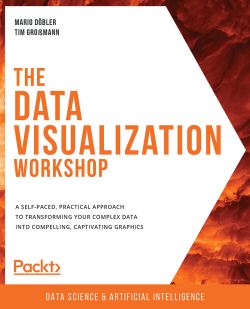# The Data Visualization Workshop

By Mario Döbler , Tim Großmann
• \$46.99 Print
• \$33.99 eBook
• \$12.99 eBook + Subscription
What do you get with a Packt Subscription?

• Constantly updated with 100+ new titles each month
• Breadth and depth in over 1,000+ technologies

Do you want to transform data into captivating images? Do you want to make it easy for your audience to process and understand the patterns, trends, and relationships hidden within your data?

The Data Visualization Workshop will guide you through the world of data visualization and help you to unlock simple secrets for transforming data into meaningful visuals with the help of exciting exercises and activities.

Starting with an introduction to data visualization, this book shows you how to first prepare raw data for visualization using NumPy and pandas operations. As you progress, you’ll use plotting techniques, such as comparison and distribution, to identify relationships and similarities between datasets. You’ll then work through practical exercises to simplify the process of creating visualizations using Python plotting libraries such as Matplotlib and Seaborn. If you’ve ever wondered how popular companies like Uber and Airbnb use geoplotlib for geographical visualizations, this book has got you covered, helping you analyze and understand the process effectively. Finally, you’ll use the Bokeh library to create dynamic visualizations that can be integrated into any web page.

By the end of this workshop, you’ll have learned how to present engaging mission-critical insights by creating impactful visualizations with real-world data.

Publication date:
July 2020
Publisher
Packt
Pages
536
ISBN
9781800568846

# 1. The Importance of Data Visualization and Data Exploration

Overview

This chapter introduces you to the basics of the statistical analysis of a dataset. We will look at basic operations for calculating the mean, median, and variance of different datasets and use NumPy and pandas to filter, sort, and shape the datasets to our requirements. The concepts we will cover will serve as a base of knowledge for the upcoming visualization chapters, in which we will work with real-world datasets. By the end of this chapter, you will be able to explain the importance of data visualization and calculate basic statistical values (such as median, mean, and variance), and use NumPy and pandas for data wrangling.

# Introduction

Unlike machines, people are usually not equipped for interpreting a large amount of information from a random set of numbers and messages in each piece of data. Out of all our logical capabilities, we understand things best through the visual processing of information. When data is represented visually, the probability of understanding complex builds and numbers increases.

Python has recently emerged as a programming language that performs well for data analysis. It has applications across data science pipelines that convert data into a usable format (such as pandas), analyzes it (such as NumPy), and extract useful conclusions from the data to represent it in a visually appealing manner (such as Matplotlib or Bokeh). Python provides data visualization libraries that can help you assemble graphical representations efficiently.

In this book, you will learn how to use Python in combination with various libraries, such as NumPy, pandas, Matplotlib, seaborn, and geoplotlib, to create impactful data visualizations using real-world data. Besides that, you will also learn about the features of different types of charts and compare their advantages and disadvantages. This will help you choose the chart type that's suited to visualizing your data.

Once we understand the basics, we can cover more advanced concepts, such as interactive visualizations and how Bokeh can be used to create animated visualizations that tell a story. Upon completing this book, you will be able to perform data wrangling, extract relevant information, and visualize your findings descriptively.

## Introduction to Data Visualization

Computers and smartphones store data such as names and numbers in a digital format. Data representation refers to the form in which you can store, process, and transmit data.

Representations can narrate a story and convey fundamental discoveries to your audience. Without appropriately modeling your information to use it to make meaningful findings, its value is reduced. Creating representations helps us achieve a more precise, more concise, and more direct perspective of information, making it easier for anyone to understand the data.

Information isn't equivalent to data. Representations are a useful apparatus to derive insights from the data. Thus, representations transform data into useful information.

## The Importance of Data Visualization

Instead of just looking at data in the columns of an Excel spreadsheet, we get a better idea of what our data contains by using visualization. For instance, it's easy to see a pattern emerge from the numerical data that's given in the following scatter plot. It shows the correlation between body mass and the maximum longevity of various animals grouped by class. There is a positive correlation between body mass and maximum longevity: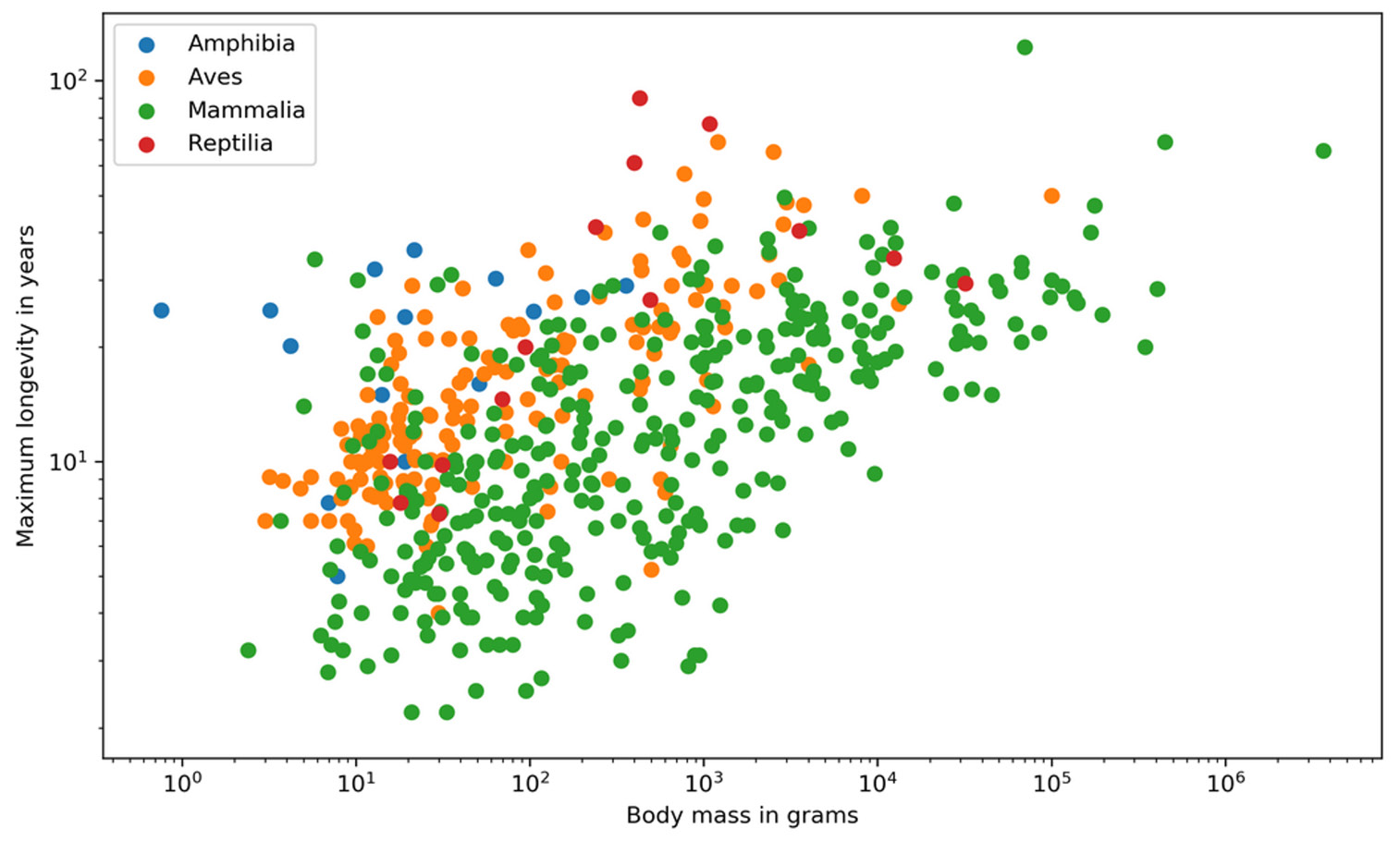Figure 1.1: A simple example of data visualization

Visualizing data has many advantages, such as the following:

• Complex data can be easily understood.
• A simple visual representation of outliers, target audiences, and futures markets can be created.
• Storytelling can be done using dashboards and animations.
• Data can be explored through interactive visualizations.

## Data Wrangling

Data wrangling is the process of transforming raw data into a suitable representation for various tasks. It is the discipline of augmenting, cleaning, filtering, standardizing, and enriching data in a way that allows it to be used in a downstream task, which in our case is data visualization.

Look at the following data wrangling process flow diagram to understand how accurate and actionable data can be obtained for business analysts to work on: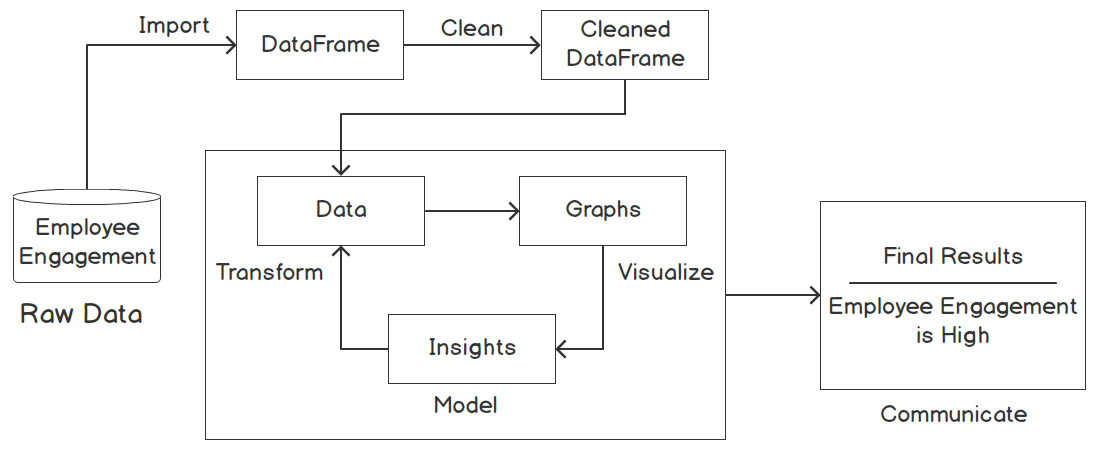Figure 1.2: Data wrangling process to measure employee engagement

In relation to the preceding figure, the following steps explain the flow of the data wrangling process:

1. First, the Employee Engagement data is in its raw form.
2. Then, the data gets imported as a DataFrame and is later cleaned.
3. The cleaned data is then transformed into graphs, from which findings can be derived.
4. Finally, we analyze this data to communicate the final results.

For example, employee engagement can be measured based on raw data gathered from feedback surveys, employee tenure, exit interviews, one-on-one meetings, and so on. This data is cleaned and made into graphs based on parameters such as referrals, faith in leadership, and scope of promotions. The percentages, that is, information derived from the graphs, help us reach our result, which is to determine the measure of employee engagement.

## Tools and Libraries for Visualization

There are several approaches to creating data visualizations. Depending on your requirements, you might want to use a non-coding tool such as Tableau, which allows you to get a good feel for your data. Besides Python, which will be used in this book, MATLAB and R are widely used in data analytics.

However, Python is the most popular language in the industry. Its ease of use and the speed at which you can manipulate and visualize data, combined with the availability of a number of libraries, make Python the best choice for data visualization.

Note

MATLAB (https://www.mathworks.com/products/matlab.html), R (https://www.r-project.org), and Tableau (https://www.tableau.com) are not part of this book; we will only cover the relevant tools and libraries for Python.

# Overview of Statistics

Statistics is a combination of the analysis, collection, interpretation, and representation of numerical data. Probability is a measure of the likelihood that an event will occur and is quantified as a number between 0 and 1.

A probability distribution is a function that provides the probability for every possible event. A probability distribution is frequently used for statistical analysis. The higher the probability, the more likely the event. There are two types of probability distributions, namely discrete and continuous.

A discrete probability distribution shows all the values that a random variable can take, together with their probability. The following diagram illustrates an example of a discrete probability distribution. If we have a six-sided die, we can roll each number between 1 and 6. We have six events that can occur based on the number that's rolled. There is an equal probability of rolling any of the numbers, and the individual probability of any of the six events occurring is 1/6: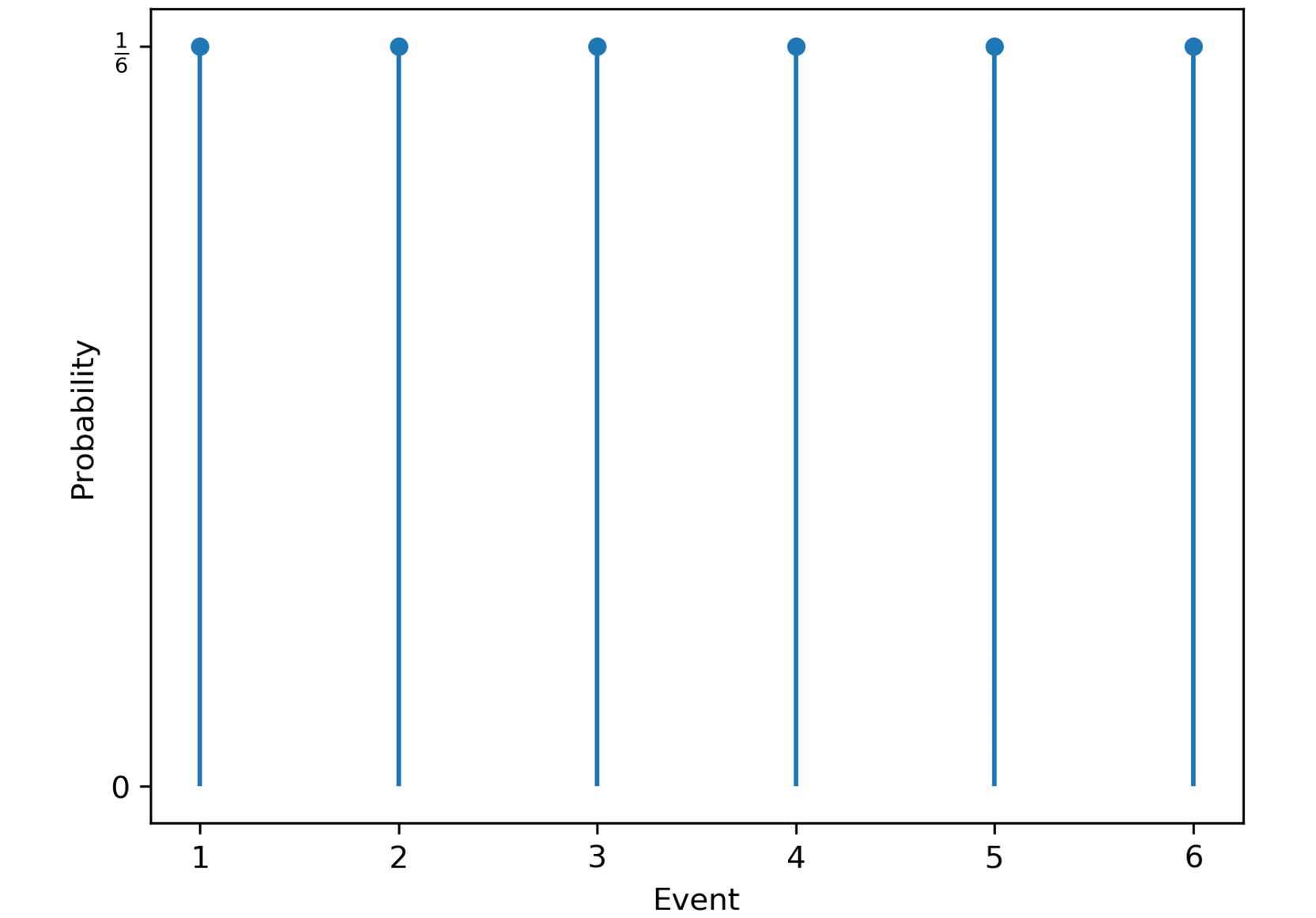Figure 1.3: Discrete probability distribution for die rolls

A continuous probability distribution defines the probabilities of each possible value of a continuous random variable. The following diagram provides an example of a continuous probability distribution. This example illustrates the distribution of the time needed to drive home. In most cases, around 60 minutes is needed, but sometimes, less time is needed because there is no traffic, and sometimes, much more time is needed if there are traffic jams: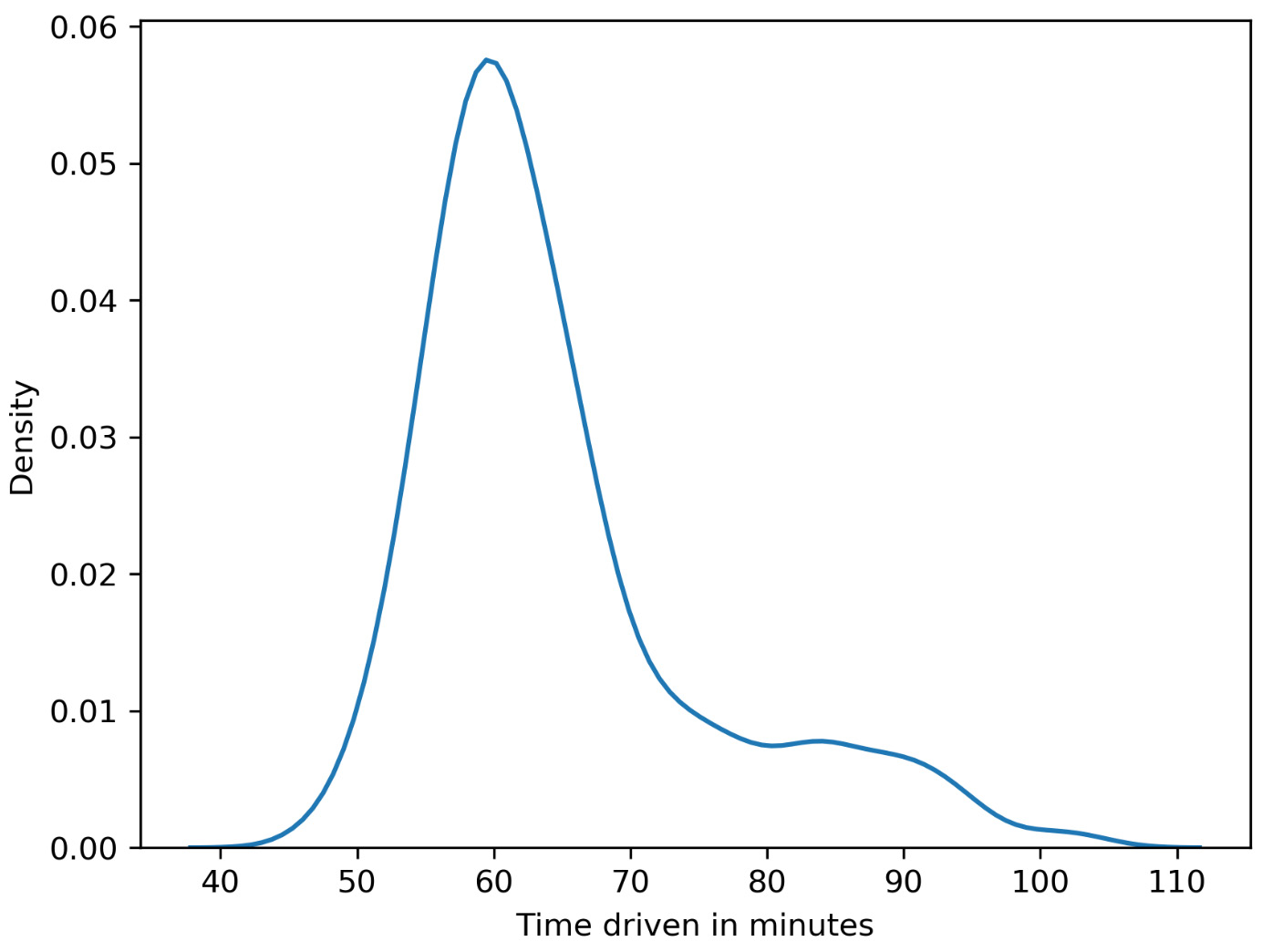Figure 1.4: Continuous probability distribution for the time taken to reach home

## Measures of Central Tendency

Measures of central tendency are often called averages and describe central or typical values for a probability distribution. We are going to discuss three kinds of averages in this chapter:

• Mean: The arithmetic average is computed by summing up all measurements and dividing the sum by the number of observations. The mean is calculated as follows:Figure 1.5: Formula for mean

• Median: This is the middle value of the ordered dataset. If there is an even number of observations, the median will be the average of the two middle values. The median is less prone to outliers compared to the mean, where outliers are distinct values in data.
• Mode: Our last measure of central tendency, the mode is defined as the most frequent value. There may be more than one mode in cases where multiple values are equally frequent.

For example, a die was rolled 10 times, and we got the following numbers: 4, 5, 4, 3, 4, 2, 1, 1, 2, and 1.

The mean is calculated by summing all the events and dividing them by the number of observations: (4+5+4+3+4+2+1+1+2+1)/10=2.7.

To calculate the median, the die rolls have to be ordered according to their values. The ordered values are as follows: 1, 1, 1, 2, 2, 3, 4, 4, 4, 5. Since we have an even number of die rolls, we need to take the average of the two middle values. The average of the two middle values is (2+3)/2=2.5.

The modes are 1 and 4 since they are the two most frequent events.

## Measures of Dispersion

Dispersion, also called variability, is the extent to which a probability distribution is stretched or squeezed.

The different measures of dispersion are as follows:

• Variance: The variance is the expected value of the squared deviation from the mean. It describes how far a set of numbers is spread out from their mean. Variance is calculated as follows:Figure 1.6: Formula for mean

• Standard deviation: This is the square root of the variance.
• Range: This is the difference between the largest and smallest values in a dataset.
• Interquartile range: Also called the midspread or middle 50%, this is the difference between the 75th and 25th percentiles, or between the upper and lower quartiles.

## Correlation

The measures we have discussed so far only considered single variables. In contrast, correlation describes the statistical relationship between two variables:

• In a positive correlation, both variables move in the same direction.
• In a negative correlation, the variables move in opposite directions.
• In zero correlation, the variables are not related.

Note

One thing you should be aware of is that correlation does not imply causation. Correlation describes the relationship between two or more variables, while causation describes how one event is caused by another. For example, consider a scenario in which ice cream sales are correlated with the number of drowning deaths. But that doesn't mean that ice cream consumption causes drowning. There could be a third variable, say temperature, that may be responsible for this correlation. Higher temperatures may cause an increase in both ice cream sales and more people engaging in swimming, which may be the real reason for the increase in deaths due to drowning.

Example

Consider you want to find a decent apartment to rent that is not too expensive compared to other apartments you've found. The other apartments (all belonging to the same locality) you found on a website are priced as follows: \$700, \$850, \$1,500, and \$750 per month. Let's calculate some values statistical measures to help us make a decision:

• The mean is (\$700 + \$850 + \$1,500 + \$750) / 4 = \$950.
• The median is (\$750 + \$850) / 2 = \$800.
• The standard deviation is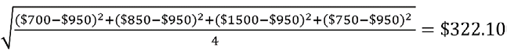.
• The range is \$1,500 - \$700 = \$800.

As an exercise, you can try and calculate the variance as well. However, note that compared with all the above values, the median value (\$800) is a better statistical measure in this case since it is less prone to outliers (the rent amount of \$1,500). Given that all apartments belong to the same locality, you can clearly see that the apartment costing \$1500 is definitely priced much higher as compared with other apartments. A simple statistical analysis helped us to narrow down our choices.

## Types of Data

It is important to understand what kind of data you are dealing with so that you can select both the right statistical measure and the right visualization. We categorize data as categorical/qualitative and numerical/quantitative. Categorical data describes characteristics, for example, the color of an object or a person's gender. We can further divide categorical data into nominal and ordinal data. In contrast to nominal data, ordinal data has an order.

Numerical data can be divided into discrete and continuous data. We speak of discrete data if the data can only have certain values, whereas continuous data can take any value (sometimes limited to a range).

Another aspect to consider is whether the data has a temporal domain – in other words, is it bound to time or does it change over time? If the data is bound to a location, it might be interesting to show the spatial relationship, so you should keep that in mind as well. The following flowchart classifies the various data types: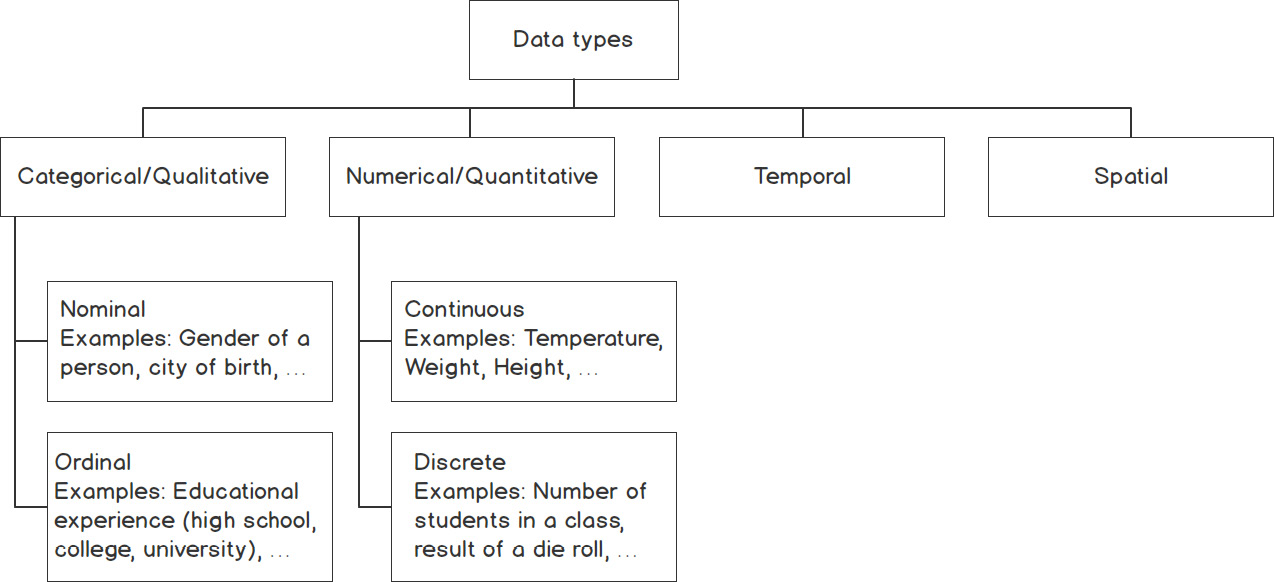Figure 1.7: Classification of types of data

## Summary Statistics

In real-world applications, we often encounter enormous datasets. Therefore, summary statistics are used to summarize important aspects of data. They are necessary to communicate large amounts of information in a compact and simple way.

We have already covered measures of central tendency and dispersion, which are both summary statistics. It is important to know that measures of central tendency show a center point in a set of data values, whereas measures of dispersion show how much the data varies.

The following table gives an overview of which measure of central tendency is best suited to a particular type of data: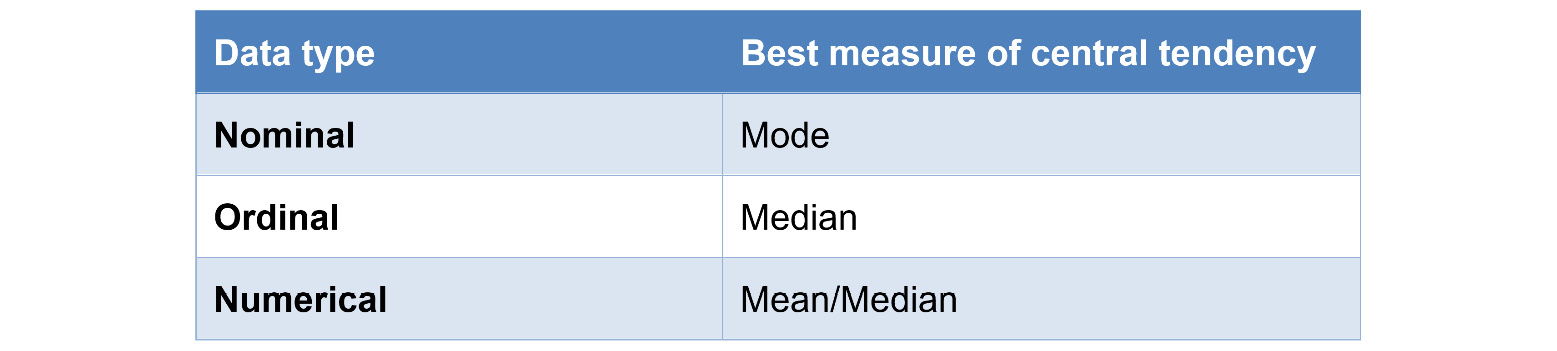Figure 1.8: Best suited measures of central tendency for different types of data

In the next section, we will learn about the NumPy library and implement a few exercises using it.

# NumPy

When handling data, we often need a way to work with multidimensional arrays. As we discussed previously, we also have to apply some basic mathematical and statistical operations on that data. This is exactly where NumPy positions itself. It provides support for large n-dimensional arrays and has built-in support for many high-level mathematical and statistical operations.

Note

Before NumPy, there was a library called Numeric. However, it's no longer used, because NumPy's signature ndarray allows the performant handling of large and high-dimensional matrices.

Ndarrays are the essence of NumPy. They are what makes it faster than using Python's built-in lists. Other than the built-in list data type, ndarrays provide a stridden view of memory (for example, `int[]` in Java). Since they are homogeneously typed, meaning all the elements must be of the same type, the stride is consistent, which results in less memory wastage and better access times.

The stride is the number of locations between the beginnings of two adjacent elements in an array. They are normally measured in bytes or in units of the size of the array elements. A stride can be larger or equal to the size of the element, but not smaller; otherwise, it would intersect the memory location of the next element.

Note

Remember that NumPy arrays have a defined data type. This means you are not able to insert strings into an integer type array. NumPy is mostly used with double-precision data types.

The following are some of the built-in methods that we will use in the exercises and activities of this chapter.

mean

NumPy provides implementations of all the mathematical operations we covered in the Overview of Statistics section of this chapter. The mean, or average, is the one we will look at in more detail in the upcoming exercise:

Note

The `#` symbol in the code snippet below denotes a code comment. Comments are added into code to help explain specific bits of logic.

```# mean value for the whole dataset
np.mean(dataset)
# mean value of the first row
np.mean(dataset)
# mean value of the whole first column
np.mean(dataset[:, 0]
# mean value of the first 10 elements of the second row
np.mean(dataset[1, 0:10])```

median

Several of the mathematical operations have the same interface. This makes them easy to interchange if necessary. The `median`, `var`, and `std` methods will be used in the upcoming exercises and activities:

```# median value for the whole dataset
np.median(dataset)
# median value of the last row using reverse indexing
np.median(dataset[-1])
# median value of values of rows >5 in the first column
np.median(dataset[5:, 0])```

Note that we can index every element from the end of our dataset as we can from the front by using reverse indexing. It's a simple way to get the last or several of the last elements of a list. Instead of `` for the first/last element, it starts with `dataset[-1]` and then decreases until `dataset[-len(dataset)]`, which is the first element in the dataset.

var

As we mentioned in the Overview of Statistics section, the variance describes how far a set of numbers is spread out from their mean. We can calculate the variance using the `var` method of NumPy:

```# variance value for the whole dataset
np.var(dataset)
# axis used to get variance per column
np.var(dataset, axis=0)
# axis used to get variance per row
np.var(dataset, axis=1)```

std

One of the advantages of the standard deviation is that it remains in the scalar system of the data. This means that the unit of the deviation will have the same unit as the data itself. The `std` method works just like the others:

```# standard deviation for the whole dataset
np.std(dataset)
# std value of values from the 2 first rows and columns
np.std(dataset[:2, :2])
# axis used to get standard deviation per row
np.std(dataset, axis=1)```

Now we will do an exercise to load a dataset and calculate the mean using these methods.

Note

All the exercises and activities in this chapter will be developed in Jupyter Notebooks. Please download the GitHub repository with all the prepared templates from https://packt.live/31USkof. Make sure you have installed all the libraries as mentioned in the preface.

## Exercise 1.01: Loading a Sample Dataset and Calculating the Mean Using NumPy

In this exercise, we will be loading the `normal_distribution.csv` dataset and calculating the mean of each row and each column in it:

1. Using the Anaconda Navigator launch either Jupyter Labs or Jupyter Notebook. In the directory of your choice, create a `Chapter01/Exercise1.01` folder.
2. Create a new Jupyter Notebook and save it as `Exercise1.01.ipynb` in the `Chapter01/Exercise1.01` folder.
3. Now, begin writing the code for this exercise as shown in the steps below. We begin with the import statements. Import `numpy` with an alias:
`import numpy as np`
4. Use the `genfromtxt` method of NumPy to load the dataset:

Note

The code snippet shown here uses a backslash ( `\` ) to split the logic across multiple lines. When the code is executed, Python will ignore the backslash, and treat the code on the next line as a direct continuation of the current line.

```dataset = \
np.genfromtxt('../../Datasets/normal_distribution.csv', \
delimiter=',')```

Note

In the preceding snippet, and for the rest of the book, we will be using a relative path to load the datasets. However, for the preceding code to work as intended, you need to follow the folder arrangement as present in this link: https://packt.live/3ftUu3P. Alternatively, you can also use the absolute path; for example, `dataset = np.genfromtxt('C:/Datasets/normal_distribution.csv', delimiter=',')`. If your Jupyter Notebook is saved in the same folder as the dataset, then you can simply use the filename: `dataset = np.genfromtxt('normal_distribution.csv', delimiter=',')`

The `genfromtxt` method helps load the data from a given text or `.csv` file. If everything works as expected, the generation should run through without any error or output.

Note

The `numpy.genfromtext` method is less efficient than the `pandas.read_csv` method. We shall refrain from going into the details of why this is the case as this explanation is beyond the scope of this text.

5. Check the data you just imported by simply writing the name of the ndarray in the next cell. Simply executing a cell that returns a value, such as an ndarray, will use Jupyter formatting, which looks nice and, in most cases, displays more information than using `print`:
```# looking at the dataset
dataset```

A section of the output resulting from the preceding code is as follows: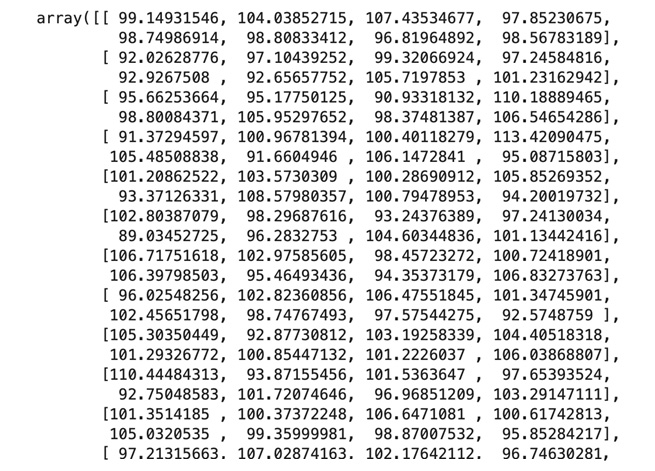Figure 1.9: The first few rows of the normal_distribution.csv file

6. Print the shape using the `dataset.shape` command to get a quick overview of our dataset. This will give us output in the form (rows, columns):
`dataset.shape`

We can also call the rows as instances and the columns as features. This means that our dataset has 24 instances and 8 features. The output of the preceding code is as follows:

`(24,8)`
7. Calculate the mean after loading and checking our dataset. The first row in a NumPy array can be accessed by simply indexing it with zero; for example, `dataset`. As we mentioned previously, NumPy has some built-in functions for calculations such as the mean. Call `np.mean()` and pass in the dataset's first row to get the result:
```# calculating the mean for the first row
np.mean(dataset)```

The output of the preceding code is as follows:

`100.177647525`
8. Now, calculate the mean of the first column by using `np.mean()` in combination with the column indexing `dataset[:, 0]`:
`np.mean(dataset[:, 0])`

The output of the preceding code is as follows:

`99.76743510416668`

Whenever we want to define a range to select from a dataset, we can use a colon, `:`, to provide start and end values for the to be selected range. If we don't provide start and end values, the default of 0 to n is used, where n is the length of the current axis.

9. Calculate the mean for every single row, aggregated in a list, using the `axis` tools of NumPy. Note that by simply passing the `axis` parameter in the `np.mean()` call, we can define the dimension our data will be aggregated on. `axis=0` is horizontal and `axis=1` is vertical. Get the result for each row by using `axis=1`:
`np.mean(dataset, axis=1)`

The output of the preceding code is as follows: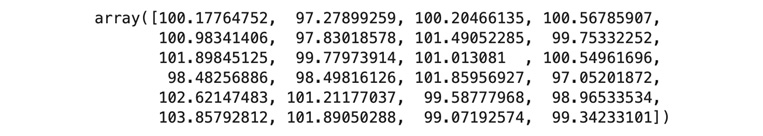Figure 1.10: Mean of the elements of each row

Get the mean of each column by using `axis=0`:

`np.mean(dataset, axis=0)`

The output of the preceding code is as follows: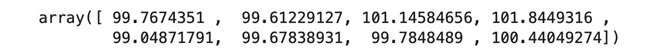Figure 1.11: Mean of elements for each column

10. Calculate the mean of the whole matrix by summing all the values we retrieved in the previous steps:
`np.mean(dataset)`

The output of the preceding code is as follows:

`100.16536917390624`

Note

To access the source code for this specific section, please refer to https://packt.live/30IkAMp.

You can also run this example online at https://packt.live/2Y4yHK1.

You are already one step closer to using NumPy in combination with plotting libraries and creating impactful visualizations. Since we've now covered the very basics and calculated the mean, it's now up to you to solve the upcoming activity.

## Activity 1.01: Using NumPy to Compute the Mean, Median, Variance, and Standard Deviation of a Dataset

In this activity, we will use the skills we've learned to import datasets and perform some basic calculations (mean, median, variance, and standard deviation) to compute our tasks.

Perform the following steps to implement this activity:

1. Open the `Activity1.01.ipynb` Jupyter Notebook from the `Chapter01` folder to do this activity. Import NumPy and give it the alias `np`.
2. Load the `normal_distribution.csv` dataset by using the `genfromtxt` method from NumPy.
3. Print a subset of the first two rows of the dataset.
4. Load the dataset and calculate the mean of the third row. Access the third row by using index 2, `dataset`.
5. Index the last element of an ndarray in the same way a regular Python list can be accessed. `dataset[:, -1]` will give us the last column of every row.
6. Get a submatrix of the first three elements of every row of the first three columns by using the double-indexing mechanism of NumPy.
7. Calculate the median for the last row of the dataset.
8. Use reverse indexing to define a range to get the last three columns. We can use `dataset[:, -3:]` here.
9. Aggregate the values along an `axis` to calculate the rows. We can use `axis=1` here.
10. Calculate the variance for each column using `axis 0`.
11. Calculate the variance of the intersection of the last two rows and the first two columns.
12. Calculate the standard deviation for the dataset.

Note

The solution for this activity can be found via this link.

You have now completed your first activity using NumPy. In the following activities, this knowledge will be consolidated further.

## Basic NumPy Operations

In this section, we will learn about basic NumPy operations such as indexing, slicing, splitting, and iterating and implement them in an exercise.

### Indexing

Indexing elements in a NumPy array, at a high level, works the same as with built-in Python lists. Therefore, we can index elements in multi-dimensional matrices:

```# index single element in outermost dimension
dataset
# index in reversed order in outermost dimension
dataset[-1]
# index single element in two-dimensional data
dataset[1, 1]
# index in reversed order in two-dimensional data
dataset[-1, -1]```

### Slicing

Slicing has also been adapted from Python's lists. Being able to easily slice parts of lists into new ndarrays is very helpful when handling large amounts of data:

```# rows 1 and 2
dataset[1:3]
# 2x2 subset of the data
dataset[:2, :2]
# last row with elements reversed
dataset[-1, ::-1]
# last 4 rows, every other element up to index 6
dataset[-5:-1, :6:2]```

### Splitting

Splitting data can be helpful in many situations, from plotting only half of your time-series data to separating test and training data for machine learning algorithms.

There are two ways of splitting your data, horizontally and vertically. Horizontal splitting can be done with the `hsplit` method. Vertical splitting can be done with the `vsplit` method:

```# split horizontally in 3 equal lists
np.hsplit(dataset, (3))
# split vertically in 2 equal lists
np.vsplit(dataset, (2))```

### Iterating

Iterating the NumPy data structures, ndarrays, is also possible. It steps over the whole list of data one after another, visiting every single element in the ndarray once. Considering that they can have several dimensions, indexing gets very complex.

The `nditer` is a multi-dimensional iterator object that iterates over a given number of arrays:

```# iterating over whole dataset (each value in each row)
for x in np.nditer(dataset):
print(x)```

The `ndenumerate` will give us exactly this index, thus returning (`0`, `1`) for the second value in the first row:

Note

The triple-quotes ( `"""` ) shown in the code snippet below are used to denote the start and end points of a multi-line code comment. Comments are added into code to help explain specific bits of logic.

```"""
iterating over the whole dataset with indices matching the
position in the dataset
"""
for index, value in np.ndenumerate(dataset):
print(index, value)```

Now, we will perform an exercise using these basic NumPy operations.

## Exercise 1.02: Indexing, Slicing, Splitting, and Iterating

In this exercise, we will use the features of NumPy to index, slice, split, and iterate ndarrays to consolidate what we've learned. A client has provided us with a dataset, `normal_distribution_splittable.csv`, wants us to confirm that the values in the dataset are closely distributed around the mean value of 100.

Note

You can obviously plot a distribution and show the spread of data, but here we want to practice implementing the aforementioned operations using the NumPy library.

Let's use the features of NumPy to index, slice, split, and iterate ndarrays.

Indexing

1. Create a new Jupyter Notebook and save it as `Exercise1.02.ipynb` in the `Chapter01/Exercise1.02` folder.
2. Import the necessary libraries:
`import numpy as np`
3. Load the `normal_distribution.csv` dataset using NumPy. Have a look at the ndarray to verify that everything works:
```dataset = np.genfromtxt('../../Datasets/'\
'normal_distribution_splittable.csv', \
delimiter=',')```

Note

As mentioned in the previous exercise, here too we have used a relative path to load the dataset. You can change the path depending on where you have saved the Jupyter Notebook and the dataset.

Remember that we need to show that our dataset is closely distributed around a mean of 100; that is, whatever value we wish to show/calculate should be around 100. For this purpose, first we will calculate the mean of the values of the second and the last row.

4. Use simple indexing for the second row, as we did in our first exercise. For a clearer understanding, all the elements of the second row are saved to a variable and then we calculate the mean of these elements:
```second_row = dataset
np.mean(second_row)```

The output of the preceding code is as follows:

`96.90038836444445`
5. Now, reverse index the last row and calculate the mean of that row. Always remember that providing a negative number as the index value will index the list from the end:
```last_row = dataset[-1]
np.mean(last_row)```

The output of the preceding code is as follows:

`100.18096645222221`

From the outputs obtained in step 4 and 5, we can say that these values indeed are close to 100. To further convince our client, we will access the first value of the first row and the last value of the second last row.

6. Index the first value of the first row using the Python standard syntax of :
```first_val_first_row = dataset
np.mean(first_val_first_row)```

The output of the preceding code is as follows:

`99.14931546`
7. Use reverse indexing to access the last value of the second last row (we want to use the combined access syntax here). Remember that `-1` means the last element:
```last_val_second_last_row = dataset[-2, -1]
np.mean(last_val_second_last_row)```

The output of the preceding code is as follows:

`101.2226037`

Note

For steps 6 and 7, even if you had not used `np.mean()`, you would have got the same values as presently shown. This is because the mean of a single value will be the value itself. You can try the above steps with the following code:

`first_val_first_row = dataset`

`first_val_first_row`

`last_val_second_last_row = dataset[-2, -1]`

`last_val_second_last_row`

From all the preceding outputs, we can confidently say that the values we obtained hover around a mean of 100. Next, we'll use slicing, splitting, and iterating to achieve our goal.

Slicing

1. Create a 2x2 matrix that starts at the second row and second column using `[1:3, 1:3]`:
```"""
slicing an intersection of 4 elements (2x2) of the
first two rows and first two columns
"""
subsection_2x2 = dataset[1:3, 1:3]
np.mean(subsection_2x2)```

The output of the preceding code is as follows:

`95.63393608250001`
2. In this task, we want to have every other element of the fifth row. Provide indexing of `::2` as our second element to get every second element of the given row:
```every_other_elem = dataset[4, ::2]
np.mean(every_other_elem)```

The output of the preceding code is as follows:

`98.35235805800001`

Introducing the second column into the indexing allows us to add another layer of complexity. The third value allows us to only select certain values (such as every other element) by providing a value of 2. This means it skips the values between and only takes each second element from the used list.

3. Reverse the elements in a slice using negative numbers:
```reversed_last_row = dataset[-1, ::-1]
np.mean(reversed_last_row)```

The output of the preceding code is as follows:

`100.18096645222222`

Splitting

1. Use the `hsplit` method to split our dataset into three equal parts:
`hor_splits = np.hsplit(dataset,(3))`

Note that if the dataset can't be split with the given number of slices, it will throw an error.

2. Split the first third into two equal parts vertically. Use the `vsplit` method to vertically split the dataset in half. It works like `hsplit`:
`ver_splits = np.vsplit(hor_splits,(2))`
3. Compare the shapes. We can see that the subset has the required half of the rows and the third half of the columns:
```print("Dataset", dataset.shape)
print("Subset", ver_splits.shape)```

The output of the preceding code is as follows:

```Dataset (24, 9)
Subset (12, 3)```

Iterating

1. Iterate over the whole dataset (each value in each row):
```curr_index = 0
for x in np.nditer(dataset):
print(x, curr_index)
curr_index += 1```

The output of the preceding code is as follows:Figure 1.12: Iterating the entire dataset

Looking at the given piece of code, we can see that the index is simply incremented with each element. This only works with one-dimensional data. If we want to index multi-dimensional data, this won't work.

2. Use the `ndenumerate` method to iterate over the whole dataset. It provides two positional values, `index` and `value`:
```for index, value in np.ndenumerate(dataset):
print(index, value)```

The output of the preceding code is as follows: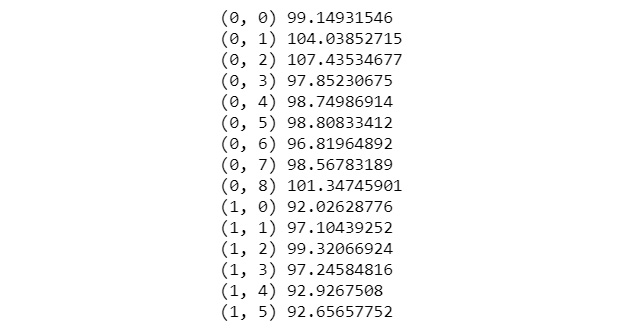Figure 1.13: Enumerating the dataset with multi-dimensional data

Notice that all the output values we obtained are close to our mean value of 100. Thus, we have successfully managed to convince our client using several NumPy methods that our data is closely distributed around the mean value of 100.

Note

To access the source code for this specific section, please refer to https://packt.live/2Neteuh.

You can also run this example online at https://packt.live/3e7K0qq.

We've already covered most of the basic data wrangling methods for NumPy. In the next section, we'll take a look at more advanced features that will give you the tools you need to get better at analyzing your data.

In this section, we will learn about advanced NumPy operations such as filtering, sorting, combining, and reshaping and then implement them in an exercise.

### Filtering

Filtering is a very powerful tool that can be used to clean up your data if you want to avoid outlier values.

In addition to the `dataset[dataset > 10]` shorthand notation, we can use the built-in NumPy `extract` method, which does the same thing using a different notation, but gives us greater control with more complex examples.

If we only want to extract the indices of the values that match our given condition, we can use the built-in `where` method. For example, `np.where(dataset > 5)` will return a list of indices of the values from the initial dataset that is bigger than 5:

```# values bigger than 10
dataset[dataset > 10]
# alternative – values smaller than 3
np.extract((dataset < 3), dataset)
# values bigger 5 and smaller 10
dataset[(dataset > 5) & (dataset < 10)]
# indices of values bigger than 5 (rows and cols)
np.where(dataset > 5)```

### Sorting

Sorting each row of a dataset can be really useful. Using NumPy, we are also able to sort on other dimensions, such as columns.

In addition, `argsort` gives us the possibility to get a list of indices, which would result in a sorted list:

```# values sorted on last axis
np.sort(dataset)
# values sorted on axis 0
np.sort(dataset, axis=0)
# indices of values in sorted list
np.argsort(dataset)```

### Combining

Stacking rows and columns onto an existing dataset can be helpful when you have two datasets of the same dimension saved to different files.

Given two datasets, we use vstack to stack `dataset_1` on top of `dataset_2`, which will give us a combined dataset with all the rows from `dataset_1`, followed by all the rows from `dataset_2`.

If we use hstack, we stack our datasets "next to each other," meaning that the elements from the first row of `dataset_1` will be followed by the elements of the first row of `dataset_2`. This will be applied to each row:

```# combine datasets vertically
np.vstack([dataset_1, dataset_2])
# combine datasets horizontally
np.hstack([dataset_1, dataset_2])
# combine datasets on axis 0
np.stack([dataset_1, dataset_2], axis=0)```

### Reshaping

Reshaping can be crucial for some algorithms. Depending on the nature of your data, it might help you to reduce dimensionality to make visualization easier:

```# reshape dataset to two columns x rows
dataset.reshape(-1, 2)
# reshape dataset to one row x columns
np.reshape(dataset, (1, -1))```

Here, `-1` is an unknown dimension that NumPy identifies automatically. NumPy will figure out the length of any given array and the remaining dimensions and will thus make sure that it satisfies the required standard.

Next, we will perform an exercise using advanced NumPy operations.

## Exercise 1.03: Filtering, Sorting, Combining, and Reshaping

This final exercise for NumPy provides some more complex tasks to consolidate our learning. It will also combine most of the previously learned methods as a recap. We'll use the filtering features of NumPy for sorting, stacking, combining, and reshaping our data:

1. Create a new Jupyter Notebook and save it as `Exercise1.03.ipynb` in the `Chapter01/Exercise1.03` folder.
2. Import the necessary libraries:
`import numpy as np`
3. Load the `normal_distribution_splittable.csv` dataset using NumPy. Make sure that everything works by having a look at the ndarray:
```dataset = np.genfromtxt('../../Datasets/'\
'normal_distribution_splittable.csv', \
delimiter=',')
dataset```
4. You will obtain the following output: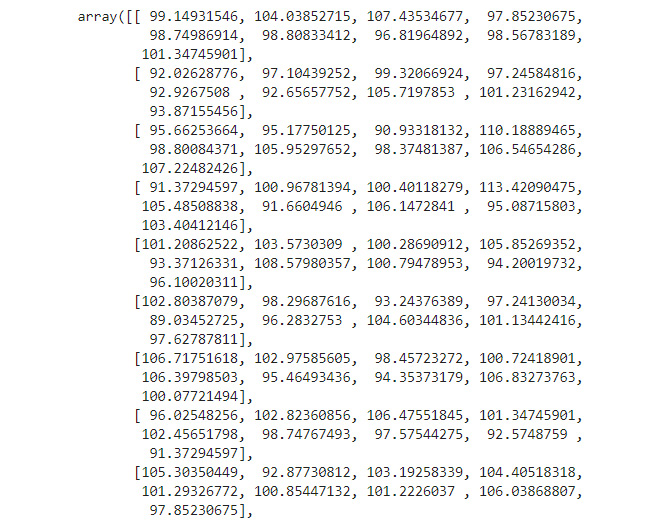Figure 1.14: Rows and columns of the dataset

Note

For ease of presentation, we have shown only a part of the output.

Filtering

1. Get values greater than 105 by supplying the condition `> 105` in the brackets:
```vals_greater_five = dataset[dataset > 105]
vals_greater_five```

You will obtain the following output: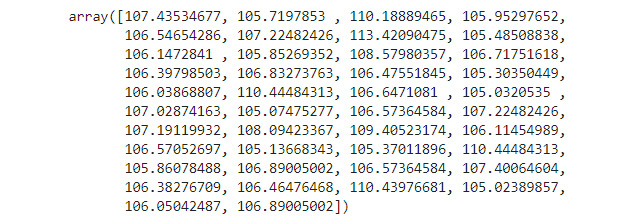Figure 1.15: Filtered dataset displaying values greater than 105

You can see in the preceding figure that all the values in the output are greater than 105.

2. Extract the values of our dataset that are between the values 90 and 95. To use more complex conditions, we might want to use the `extract` method of NumPy:
```vals_between_90_95 = np.extract((dataset > 90) \
& (dataset < 95), dataset)
vals_between_90_95```

You will obtain the following output: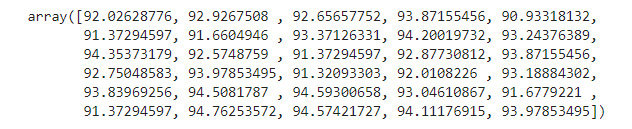Figure 1.16: Filtered dataset displaying values between 90 and 95

The preceding output clearly shows that only values lying between 90 and 95 are printed.

3. Use the `where` method to get the indices of values that have a delta of less than 1; that is, [individual value] – 100 should be less than 1. Use those indices (`row, col)` in a list comprehension and print them out:
```rows, cols = np.where(abs(dataset - 100) < 1)
one_away_indices = [[rows[index], \
cols[index]] for (index, _) \
in np.ndenumerate(rows)]
one_away_indices```

The `where` method from NumPy allows us to get `indices (rows, cols)` for each of the matching values.

Observe the following truncated output: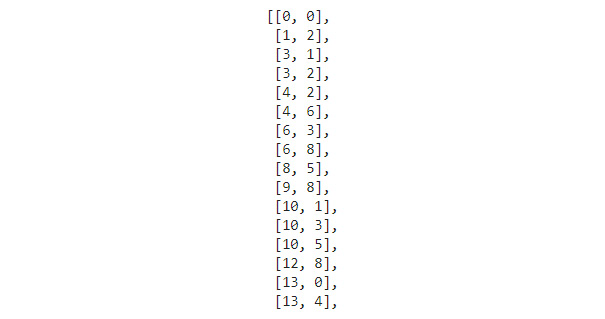Figure 1.17: Indices of the values that have a delta of less than 1

Let us confirm if we indeed obtained the right indices. The first set of indices `0,0` refer to the very first value in the output shown in Figure 1.14. Indeed, this is the correct value as `abs (99.14931546 – 100) < 1`. We can quickly check this for a couple of more values and conclude that indeed the code has worked as intended.

Note

List comprehensions are Python's way of mapping over data. They're a handy notation for creating a new list with some operation applied to every element of the old list.

For example, if we want to double the value of every element in this list, `list = [1, 2, 3, 4, 5]`, we would use list comprehensions like this: `doubled_list=[x*x for x in list]`. This gives us the following list: `[1, 4, 9, 16, 25]`. To get a better understanding of list comprehensions, please visit https://docs.python.org/3/tutorial/datastructures.html#list-comprehensions.

Sorting

1. Sort each row in our dataset by using the `sort` method:
```row_sorted = np.sort(dataset)
row_sorted```

As described before, by default, the last axis will be used. In a two-dimensional dataset, this is axis 1 which represents the rows. So, we can omit the `axis=1` argument in the `np.sort` method call. You will obtain the following output: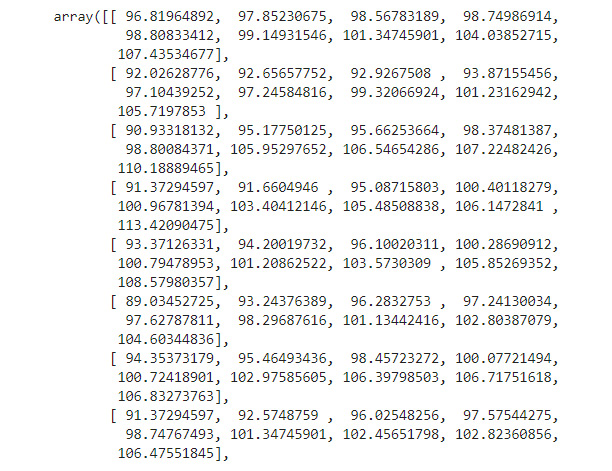Figure 1.18: Dataset with sorted rows

Compare the preceding output with that in Figure 1.14. What do you observe? The values along the rows have been sorted in an ascending order as expected.

2. With multi-dimensional data, we can use the `axis` parameter to define which dataset should be sorted. Use the `0` axes to sort the values by column:
```col_sorted = np.sort(dataset, axis=0)
col_sorted```

A truncated version of the output is as follows: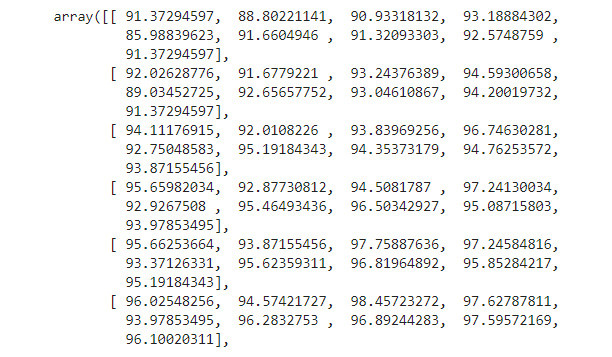Figure 1.19: Dataset with sorted columns

As expected, the values along the columns (91.37294597, 91.02628776, 94.11176915, and so on) are now sorted in an ascending order.

3. Create a sorted index list and use fancy indexing to get access to sorted elements easily. To keep the order of our dataset and obtain only the values of a sorted dataset, we will use `argsort`:
```index_sorted = np.argsort(dataset)
dataset[index_sorted]```

The output is as follows: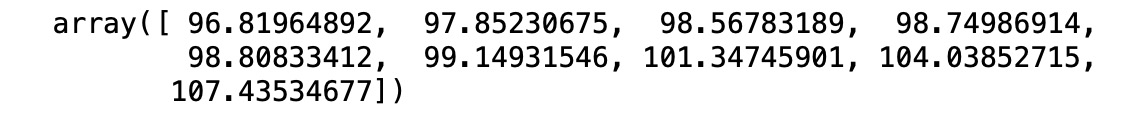Figure 1.20: First row with sorted values from argsort

As can be seen from the preceding output, we have obtained the first row with sorted values.

Combining

4. Use the combining features to add the second half of the first column back together, add the second column to our combined dataset, and add the third column to our combined dataset.
```thirds = np.hsplit(dataset, (3))
halfed_first = np.vsplit(thirds, (2))
halfed_first```

The output of the preceding code is as follows: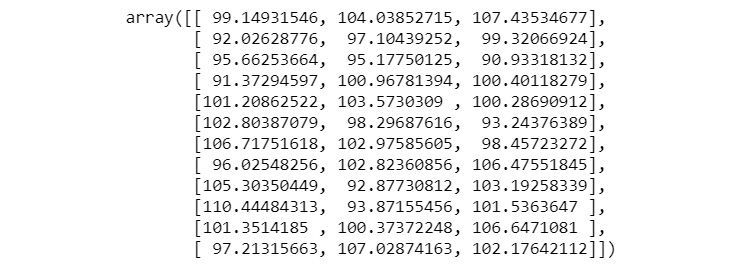Figure 1.21: Splitting the dataset

5. Use `vstack` to vertically combine the `halfed_first` datasets:
```first_col = np.vstack([halfed_first, halfed_first])
first_col```

The output of the preceding code is as follows: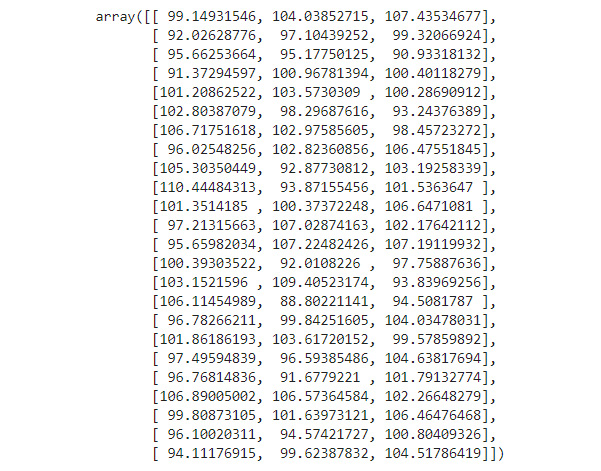Figure 1.22: Vertically combining the dataset

After stacking the second half of our split dataset, we have one-third of our initial dataset stacked together again. Now, we want to add the other two remaining datasets to our `first_col` dataset.

6. Use the `hstack` method to combine our already combined `first_col` with the second of the three split datasets:
```first_second_col = np.hstack([first_col, thirds])
first_second_col```

A truncated version of the output resulting from the preceding code is as follows: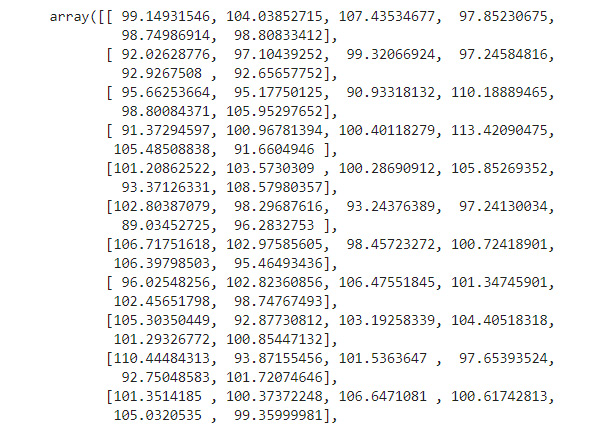Figure 1.23: Horizontally combining the dataset

7. Use `hstack` to combine the last one-third column with our dataset. This is the same thing we did with our second-third column in the previous step:
```full_data = np.hstack([first_second_col, thirds])
full_data```

A truncated version of the output resulting from the preceding code is as follows: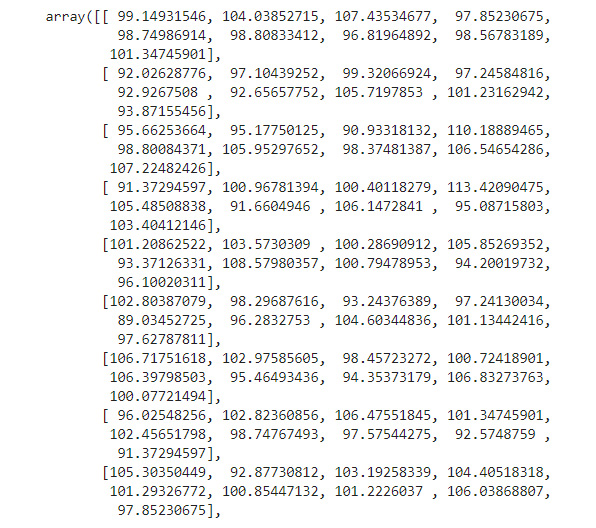Figure 1.24: The complete dataset

Reshaping

1. Reshape our dataset into a single list using the `reshape` method:
```single_list = np.reshape(dataset, (1, -1))
single_list```

A truncated version of the output resulting from the preceding code is as follows: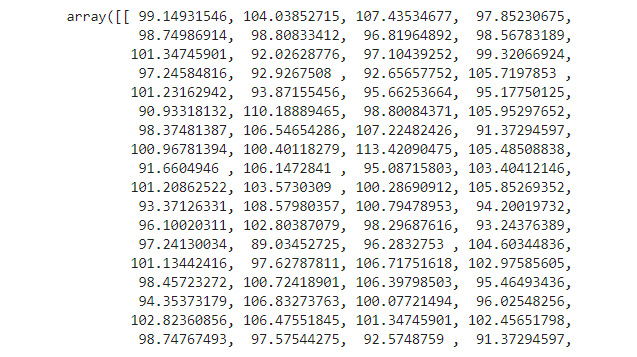Figure 1.25: Reshaped dataset

2. Provide a `-1` for the dimension. This tells NumPy to figure the dimension out itself:
```# reshaping to a matrix with two columns
two_col_dataset = dataset.reshape(-1, 2)
two_col_dataset```

A truncated version of the output resulting from the preceding code is as follows: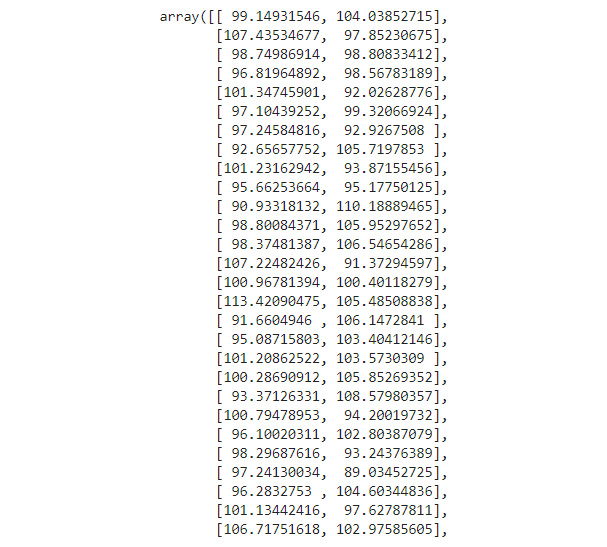Figure 1.26: The dataset in a two-column format

Note

To access the source code for this specific section, please refer to https://packt.live/2YD4AZn.

You can also run this example online at https://packt.live/3e6F7Ol.

You have now used many of the basic operations that are needed so that you can analyze a dataset. Next, we will be learning about pandas, which will provide several advantages when working with data that is more complex than simple multi-dimensional numerical data. pandas also support different data types in datasets, meaning that we can have columns that hold strings and others that have numbers.

NumPy, as you've seen, has some powerful tools. Some of them are even more powerful when combined with pandas DataFrames.

# pandas

The pandas Python library provides data structures and methods for manipulating different types of data, such as numerical and temporal data. These operations are easy to use and highly optimized for performance.

Data formats, such as CSV and JSON, and databases can be used to create DataFrames. DataFrames are the internal representations of data and are very similar to tables but are more powerful since they allow you to efficiently apply operations such as multiplications, aggregations, and even joins. Importing and reading both files and in-memory data is abstracted into a user-friendly interface. When it comes to handling missing data, pandas provide built-in solutions to clean up and augment your data, meaning it fills in missing values with reasonable values.

Integrated indexing and label-based slicing in combination with fancy indexing (what we already saw with NumPy) make handling data simple. More complex techniques, such as reshaping, pivoting, and melting data, together with the possibility of easily joining and merging data, provide powerful tooling so that you can handle your data correctly.

If you're working with time-series data, operations such as date range generation, frequency conversion, and moving window statistics can provide an advanced interface for data wrangling.

Note

The installation instructions for pandas can be found here: https://pandas.pydata.org/. The latest version is v0.25.3 (used in this book); however, every v0.25.x should be suitable.

## Advantages of pandas over NumPy

The following are some of the advantages of pandas:

• High level of abstraction: pandas have a higher abstraction level than NumPy, which gives it a simpler interface for users to interact with. It abstracts away some of the more complex concepts, such as high-performance matrix multiplications and joining tables, and makes it easier to use and understand.
• Less intuition: Many methods, such as joining, selecting, and loading files, are used without much intuition and without taking away much of the powerful nature of pandas.
• Faster processing: The internal representation of DataFrames allows faster processing for some operations. Of course, this always depends on the data and its structure.
• Easy DataFrame design: DataFrames are designed for operations with and on large datasets.

The following are some of the disadvantages of pandas:

• Less applicable: Due to its higher abstraction, it's generally less applicable than NumPy. Especially when used outside of its scope, operations can get complex.
• More disk space: Due to the internal representation of DataFrames and the way pandas trades disk space for a more performant execution, the memory usage of complex operations can spike.
• Performance problems: Especially when doing heavy joins, which is not recommended, memory usage can get critical and might lead to performance problems.
• Hidden complexity: Less experienced users often tend to overuse methods and execute them several times instead of reusing what they've already calculated. This hidden complexity makes users think that the operations themselves are simple, which is not the case.

Note

Now, we will do an exercise to load a dataset and calculate the mean using pandas.

## Exercise 1.04 Loading a Sample Dataset and Calculating the Mean using Pandas

In this exercise, we will be loading the `world_population.csv` dataset and calculating the mean of some rows and columns. Our dataset holds the yearly population density for every country. Let's use pandas to perform this exercise:

1. Create a new Jupyter Notebook and save it as `Exercise1.04.ipynb` in the `Chapter01/Exercise1.04` folder.
2. Import the `pandas` libraries:
`import pandas as pd`
3. Use the `read_csv` method to load the aforementioned dataset. We want to use the first column, containing the country names, as our index. We will use the `index_col` parameter for that:
```dataset = \
index_col=0)```
4. Now, check the data you just imported by simply writing the name of the dataset in the next cell. pandas uses a data structure called DataFrames. Print some of the rows. To avoid filling the screen, use the pandas `head()` method:
`dataset.head()`

The output of the preceding code is as follows: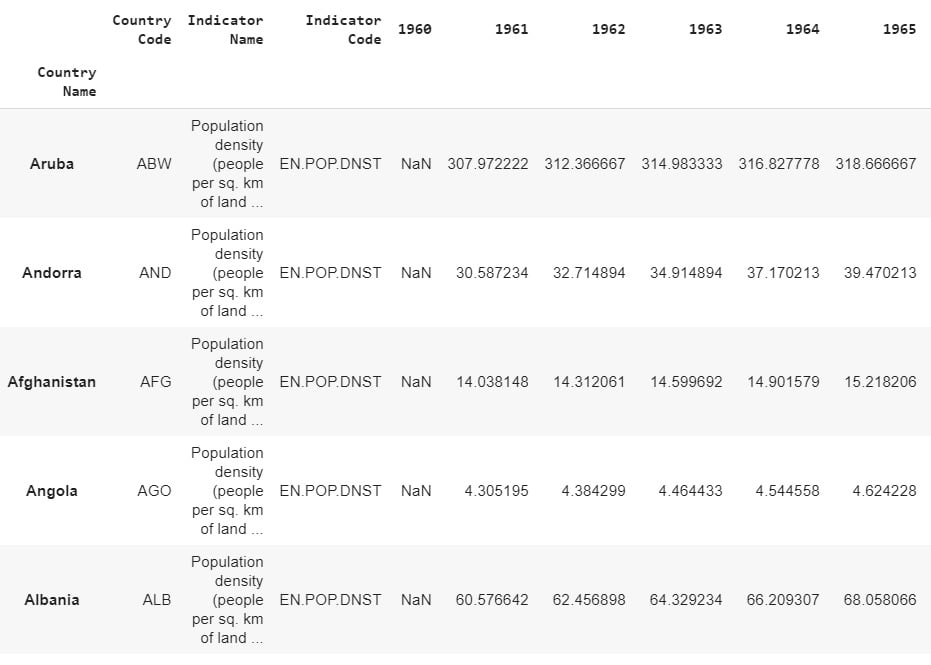Figure 1.27: The first five rows of our dataset

Both `head()` and `tail()` let you provide a number, `n`, as a parameter, which describes how many rows should be returned.

Note

Simply executing a cell that returns a value such as a DataFrame will use Jupyter formatting, which looks nicer and, in most cases, displays more information than using `print`.

5. Print out the shape of the dataset to get a quick overview using the `dataset.shape` command. This works the same as it does with NumPy ndarrays. It will give us the output in the form `(rows, columns)`:
`dataset.shape`

The output of the preceding code is as follows:

`(264, 60)`
6. Index the column with the year 1961. pandas DataFrames have built-in functions for calculations, such as the `mean`. This means we can simply call `dataset.mean()` to get the result.

The printed output should look as follows:

`dataset["1961"].mean()`

The output of the preceding code is as follows:

`176.91514132840555`
7. Check the difference in population density over the years by repeating the previous step with the column for the year 2015 (the population more than doubled in the given time range):
```# calculating the mean for 2015 column
dataset["2015"].mean()```

The output of the preceding code is as follows:

`368.70660104001837`
8. To get the mean for every single country (row), we can make use of pandas `axis` tools. Use the `mean()` method on the dataset on `axis=1`, meaning all the rows, and return the first 10 rows using the `head()` method:
`dataset.mean(axis=1).head(10)`

The output of the preceding code is as follows: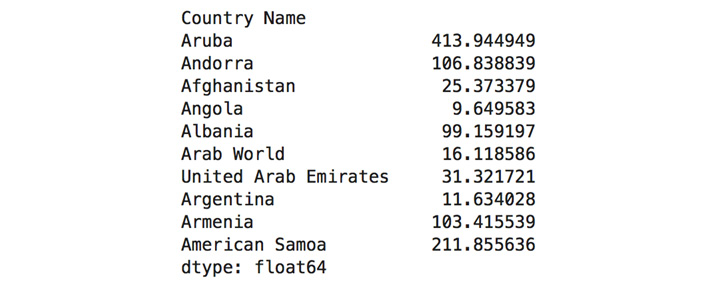Figure 1.28: Mean of elements in the first 10 countries (rows)

9. Get the mean for each column and return the last 10 entries:
`dataset.mean(axis=0).tail(10)`

The output of the preceding code is as follows: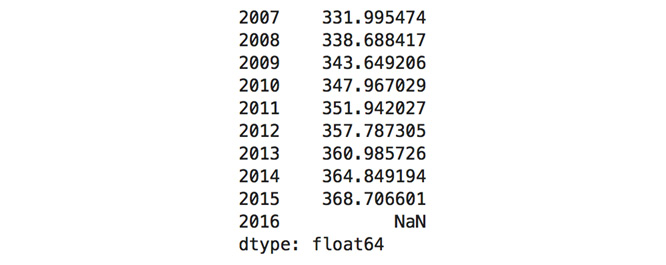Figure 1.29: Mean of elements for the last 10 years (columns)

10. Calculate the mean of the whole DataFrame:
```# calculating the mean for the whole matrix
dataset.mean()```

The output of the preceding code is as follows: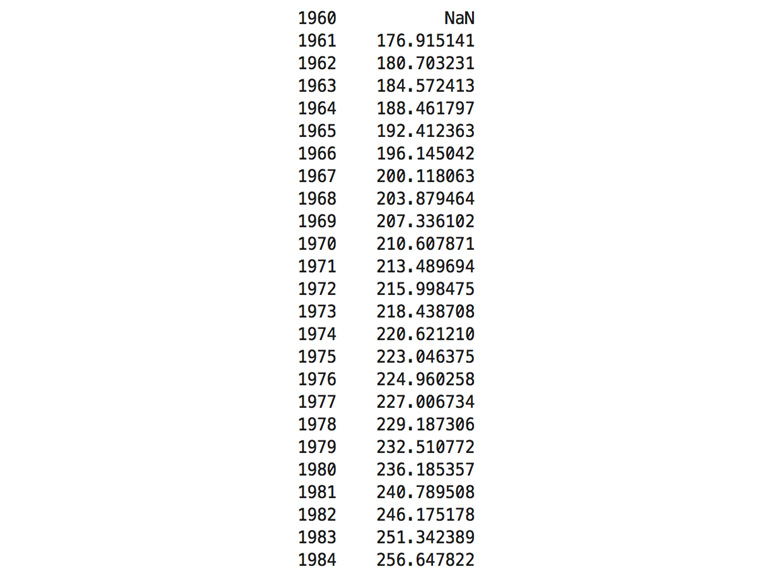Figure 1.30: Mean of elements for each column

Since pandas DataFrames can have different data types in each column, aggregating this value on the whole dataset out of the box makes no sense. By default, `axis=0` will be used, which means that this will give us the same result as the cell prior to this.

Note

To access the source code for this specific section, please refer to https://packt.live/37z3Us1.

You can also run this example online at https://packt.live/2Bb0ks8.

We've now seen that the interface of pandas has some similar methods to NumPy, which makes it really easy to understand. We have now covered the very basics, which will help you solve the first exercise using pandas. In the following exercise, you will consolidate your basic knowledge of pandas and use the methods you just learned to solve several computational tasks.

## Exercise 1.05: Using pandas to Compute the Mean, Median, and Variance of a Dataset

In this exercise, we will take the previously learned skills of importing datasets and basic calculations and apply them to solve the tasks of our first exercise using pandas.

Let's use pandas features such as `mean`, `median`, and `variance` to make some calculations on our data:

1. Create a new Jupyter Notebook and save it as `Exercise1.05.ipynb` in the `Chapter01/Exercise1.05` folder.
2. Import the necessary libraries:
`import pandas as pd`
3. Use the `read_csv` method to load the aforementioned dataset and use the `index_col` parameter to define the first column as our index:
```dataset = \
index_col=0)```
4. Print the first two rows of our dataset:
`dataset[0:2]`

The output of the preceding code is as follows: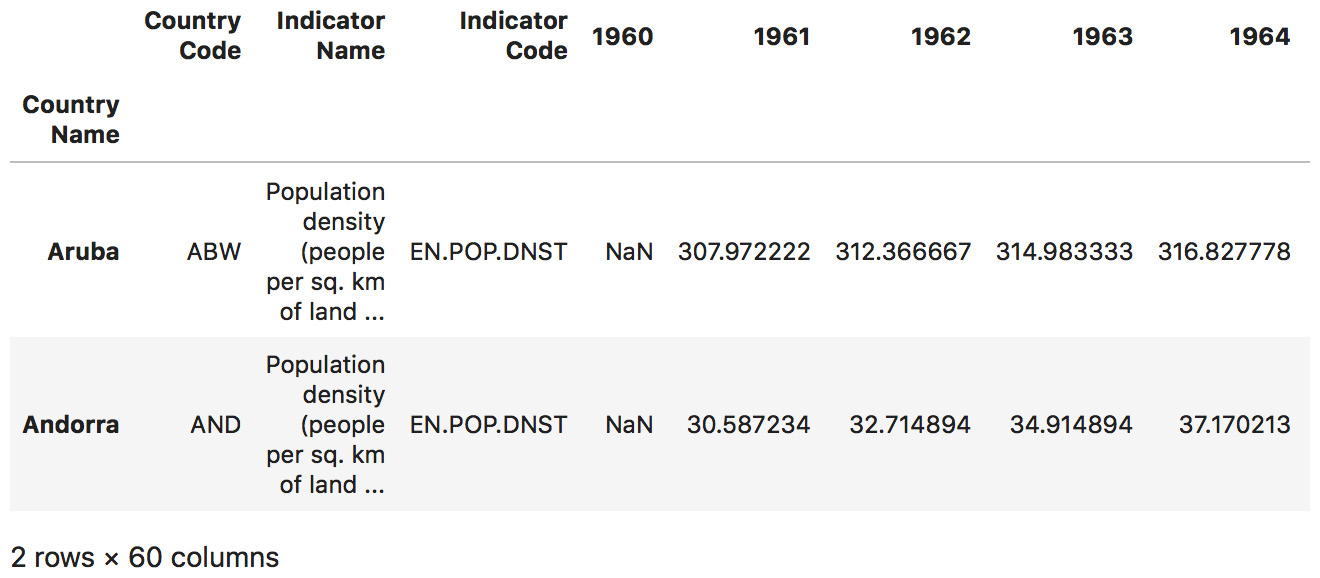Figure 1.31: The first two rows, printed

5. Now, index the third row by using `dataset.iloc[]`. Use the `axis` parameter to get the mean of the country rather than the yearly column:
`dataset.iloc[].mean(axis=1)`

The output of the preceding code is as follows:Figure 1.32: Calculating the mean of the third row

6. Index the last element of the DataFrame using `-1` as the index for the `iloc()` method:
`dataset.iloc[[-1]].mean(axis=1)`

The output of the preceding code is as follows:Figure 1.33: Calculating the mean of the last row

7. Calculate the mean value of the values labeled as Germany using `loc`, which works based on the index column:
`dataset.loc[["Germany"]].mean(axis=1)`

The output of the preceding code is as follows:Figure 1.34: Indexing a country and calculating the mean of Germany

8. Calculate the median value of the last row by using reverse indexing and `axis=1` to aggregate the values in the row:
`dataset.iloc[[-1]].median(axis=1)`

The output of the preceding code is as follows:Figure 1.35: Usage of the median method on the last row

9. Use reverse indexing to get the last three columns with `dataset[-3:]` and calculate the median for each of them:
`dataset[-3:].median(axis=1)`

The output of the preceding code is as follows:Figure 1.36: Median of the last three columns

10. Calculate the median population density values for the first 10 countries of the list using the `head` and `median` methods:
`dataset.head(10).median(axis=1)`

The output of the preceding code is as follows: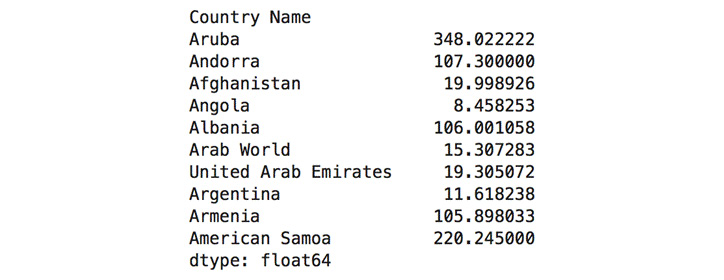Figure 1.37: Usage of the axis to calculate the median of the first 10 rows

When handling larger datasets, the order in which methods are executed matters. Think about what `head(10)` does for a moment. It simply takes your dataset and returns the first 10 rows in it, cutting down your input to the `mean()` method drastically.

The last method we'll cover here is the variance. pandas provide a consistent API, which makes it easy to use.

11. Calculate the variance of the dataset and return only the last five columns:
`dataset.var().tail()`

The output of the preceding code is as follows:Figure 1.38: Variance of the last five columns

12. Calculate the mean for the year 2015 using both NumPy and pandas separately:
```# NumPy pandas interoperability
import numpy as np
print("pandas", dataset["2015"].mean())
print("numpy", np.mean(dataset["2015"]))```

The output of the preceding code is as follows: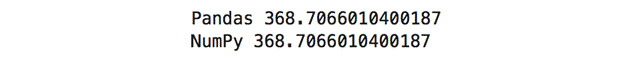Figure 1.39: Using NumPy's mean method with a pandas DataFrame

Note

To access the source code for this specific section, please refer to https://packt.live/2N7E2Kh.

You can also run this example online at https://packt.live/2Y3B2Fa.

This exercise of how to use NumPy's `mean` method with a pandas DataFrame shows that, in some cases, NumPy has better functionality. However, the DataFrame format of pandas is more applicable, so we combine both libraries to get the best out of both.

You've completed your first exercise with pandas, which showed you some of the similarities, and also differences when working with NumPy and pandas. In the following exercise, this knowledge will be consolidated. You'll also be introduced to more complex features and methods of pandas.

## Basic Operations of pandas

In this section, we will learn about the basic pandas operations, such as indexing, slicing, and iterating, and implement them with an exercise.

### Indexing

Indexing with pandas is a bit more complex than with NumPy. We can only access columns with a single bracket. To use the indices of the rows to access them, we need the `iloc` method. If we want to access them with `index_col` (which was set in the `read_csv` call), we need to use the `loc` method:

```# index the 2000 col
dataset["2000"]
# index the last row
dataset.iloc[-1]
# index the row with index Germany
dataset.loc["Germany"]
# index row Germany and column 2015
dataset[["2015"]].loc[["Germany"]]```

### Slicing

Slicing with pandas is even more powerful. We can use the default slicing syntax we've already seen with NumPy or use multi-selection. If we want to slice different rows or columns by name, we can simply pass a list into the brackets:

```# slice of the first 10 rows
dataset.iloc[0:10]
# slice of rows Germany and India
dataset.loc[["Germany", "India"]]
# subset of Germany and India with years 1970/90
dataset.loc[["Germany", "India"]][["1970", "1990"]]```

### Iterating

Iterating DataFrames is also possible. Considering that they can have several `dimensions` and `dtypes`, the indexing is very high level and iterating over each row has to be done separately:

```# iterating the whole dataset
for index, row in dataset.iterrows():
print(index, row)```

### Series

A pandas Series is a one-dimensional labeled array that is capable of holding any type of data. We can create a Series by loading datasets from a `.csv` file, Excel spreadsheet, or SQL database. There are many different ways to create them, such as the following:

• NumPy arrays:
```# import pandas
import pandas as pd
# import numpy
import numpy as np
# creating a numpy array
numarr = np.array(['p','y','t','h','o','n'])
ser = pd.Series(numarr)
print(ser)```
• pandas lists:
```# import pandas
import pandas as pd
# creating a pandas list
plist = ['p','y','t','h','o','n']
ser = pd.Series(plist)
print(ser)```

Now, we will use basic pandas operations in an exercise.

## Exercise 1.06: Indexing, Slicing, and Iterating Using pandas

In this exercise, we will use the previously discussed pandas features to index, slice, and iterate DataFrames using pandas Series. To derive some insights from our dataset, we need to be able to explicitly index, slice, and iterate our data. For example, we can compare several countries in terms of population density growth.

Let's use the indexing, slicing, and iterating operations to display the population density of Germany, Singapore, United States, and India for years 1970, 1990, and 2010.

Indexing

1. Create a new Jupyter Notebook and save it as `Exercise1.06.ipynb` in the `Chapter01/Exercise1.06` folder.
2. Import the necessary libraries:
`import pandas as pd`
3. Use the `read_csv` method to load the `world_population.csv` dataset and use the first column, (containing the country names) as our index using the `index_col` parameter:
```dataset = \
index_col=0)```
4. Index the row with the `index_col` `"United States"` using the `loc` method:
`dataset.loc[["United States"]].head()`

The output of the preceding code is as follows: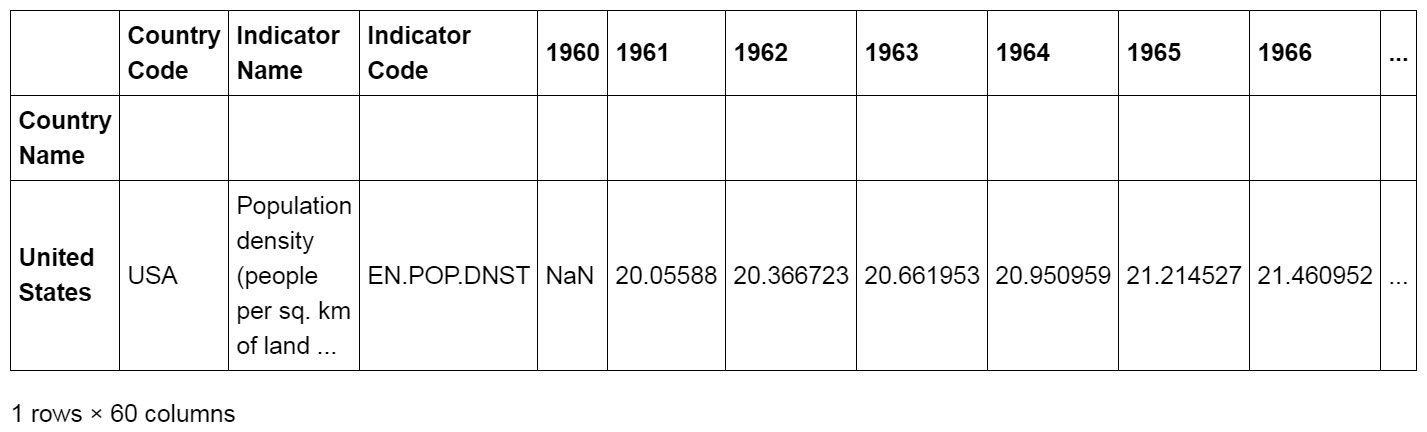Figure 1.40: A few columns from the output showing indexing United States with the loc method

5. Use reverse indexing in pandas to index the second to last row using the `iloc` method:
`dataset.iloc[[-2]]`

The output of the preceding code is as follows: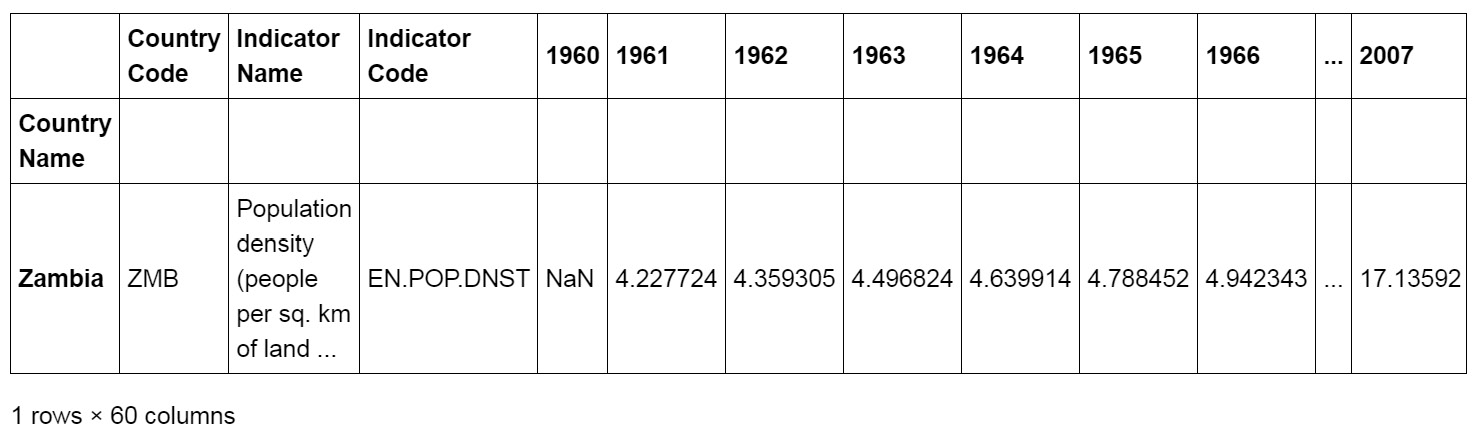Figure 1.41: Indexing the second to last row

6. Columns are indexed using their header. This is the first line of the CSV file. Index the column with the header of `2000` as a Series:
`dataset["2000"].head()`

The output of the preceding code is as follows:Figure 1.42: Indexing all 2000 columns

Remember, the `head()` method simply returns the first five rows.

7. First, get the data for the year 2000 as a DataFrame and then select India using the `loc()` method using chaining:
`dataset[["2000"]].loc[["India"]]`

The output of the preceding code is as follows:Figure 1.43: Getting the population density of India in 2000

Since the double brackets notation returns a DataFrame once again, we can chain method calls to get distinct elements.

8. Use the single brackets notation to get the distinct value for the population density of India in 2000:
`dataset["2000"].loc["India"]`

If we want to only retrieve a Series object, we must replace the double brackets with single ones. The output of the preceding code is as follows:

`354.326858357522`

Slicing

1. Create a slice with the rows 2 to 5 using the `iloc()` method again:
```# slicing countries of rows 2 to 5
dataset.iloc[1:5]```

The output of the preceding code is as follows: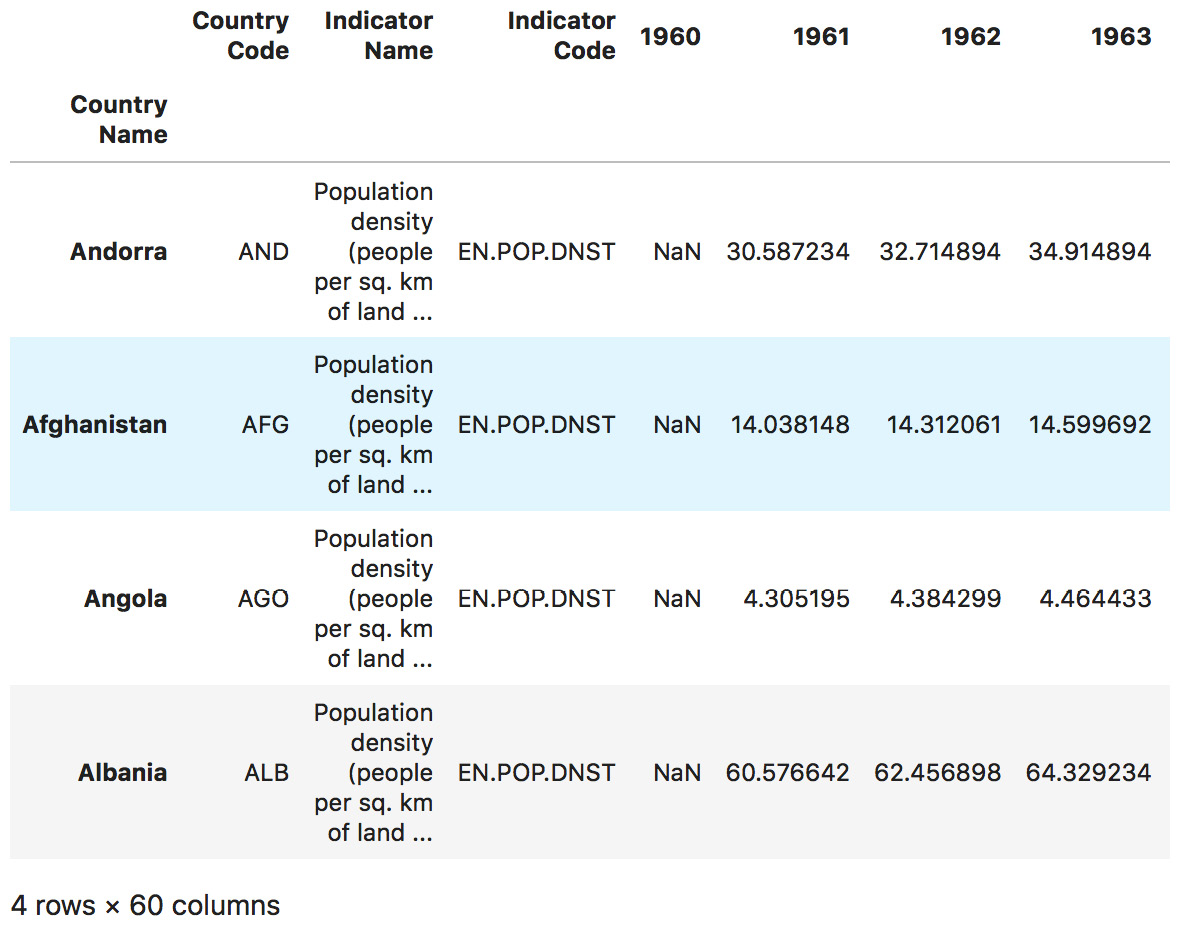Figure 1.44: The countries in rows 2 to 5

2. Use the `loc()` method to access several rows in the DataFrame and use the nested brackets to provide a list of elements. Slice the dataset to get the rows for Germany, Singapore, United States, and India:
`dataset.loc[["Germany", "Singapore", "United States", "India"]]`

The output of the preceding code is as follows: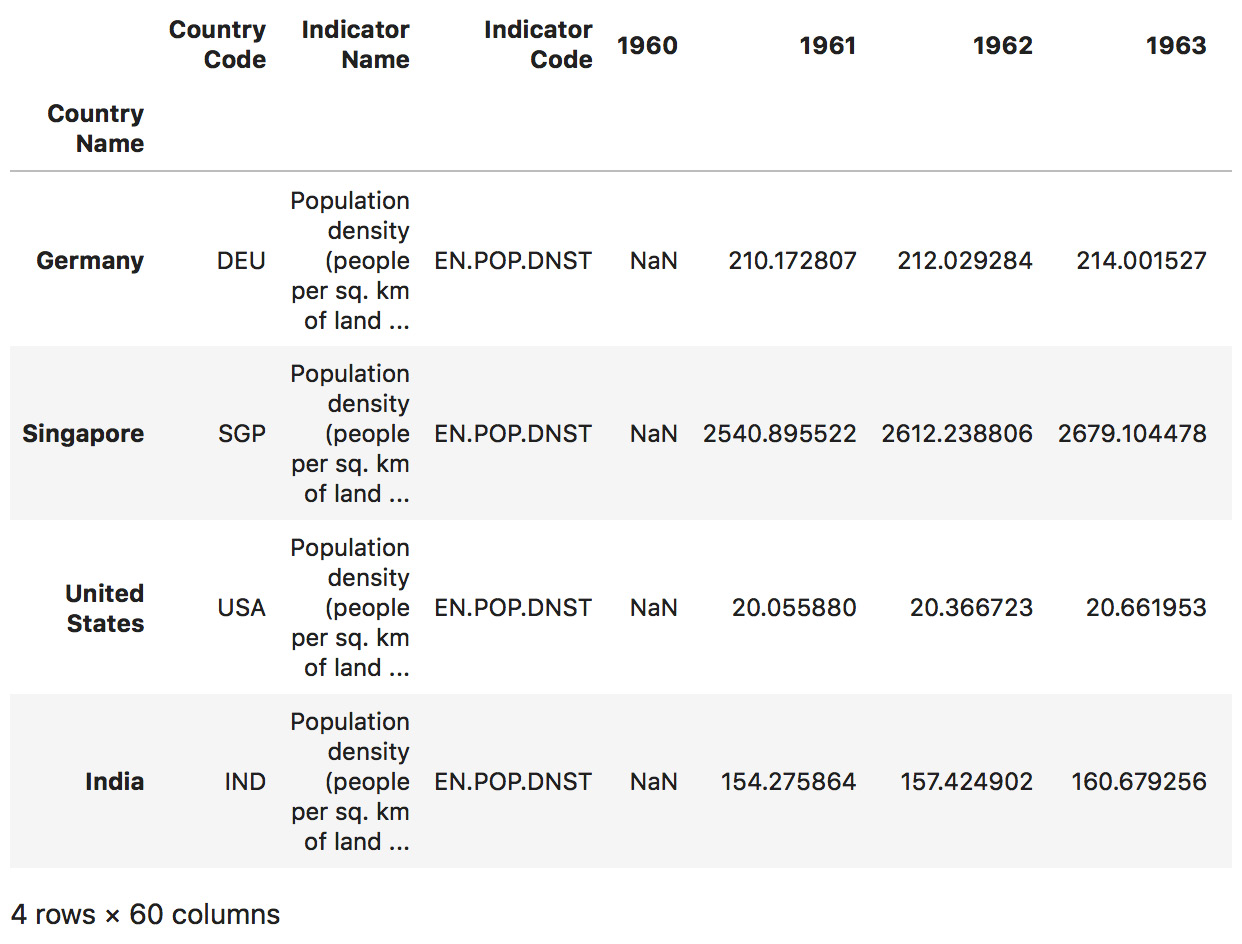Figure 1.45: Slicing Germany, Singapore, United States, and India

3. Use chaining to get the rows for Germany, Singapore, United States, and India and return only the values for the years 1970, 1990, and 2010. Since the double bracket queries return new DataFrames, we can chain methods and therefore access distinct subframes of our data:
```country_list = ["Germany", "Singapore", "United States", "India"]
dataset.loc[country_list][["1970", "1990", "2010"]]```

The output of the preceding code is as follows: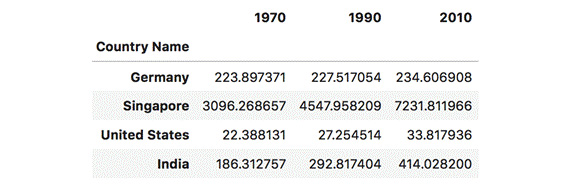Figure 1.46: Slices some of the countries and their population density for 1970, 1990, and 2010

Iterating

1. Iterate our dataset and print out the countries up until `Angola` using the `iterrows()` method. The index will be the name of our row, and the row will hold all the columns:
```for index, row in dataset.iterrows():
# only printing the rows until Angola
if index == 'Angola':
break
print(index, '\n', \
row[["Country Code", "1970", "1990", "2010"]], '\n')```

The output of the preceding code is as follows: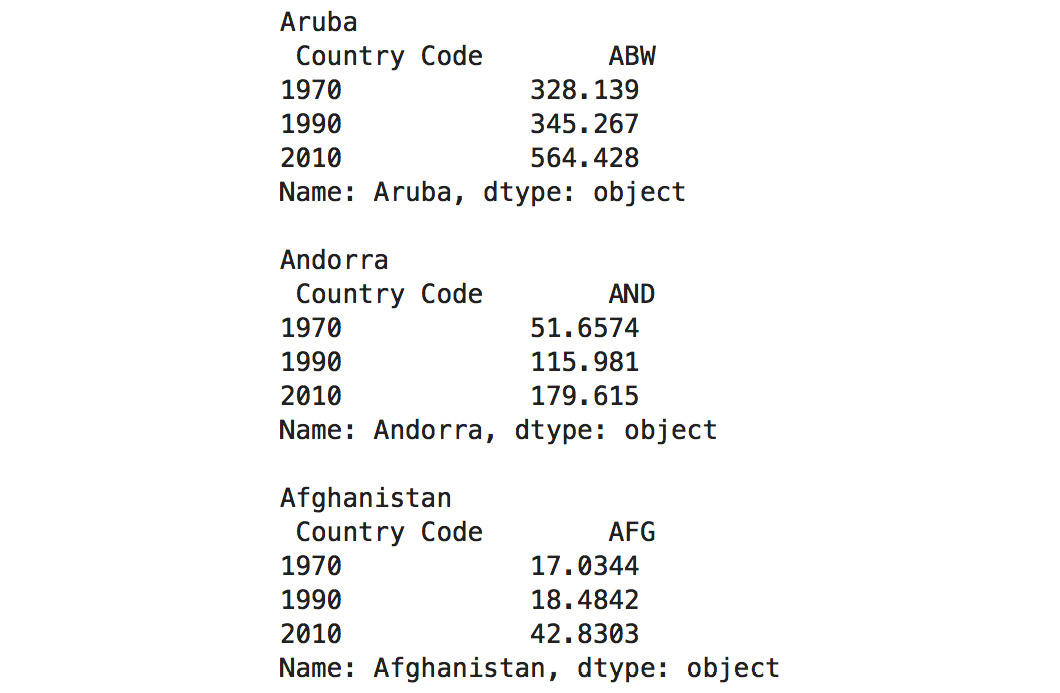Figure 1.47: Iterating all countries until Angola

Note

To access the source code for this specific section, please refer to https://packt.live/2YKqHNM.

You can also run this example online at https://packt.live/2YD56Xo.

We've already covered most of the underlying data wrangling methods using pandas. In the next exercise, we'll take a look at more advanced features such as filtering, sorting, and reshaping to prepare you for the next chapter.

In this section, we will learn about some advanced pandas operations such as filtering, sorting, and reshaping and implement them in an exercise.

### Filtering

Filtering in pandas has a higher-level interface than NumPy. You can still use the simple brackets-based conditional filtering. However, you're also able to use more complex queries, for example, filter rows based on labels using likeness, which allows us to search for a substring using the `like` argument and even full regular expressions using `regex`:

```# only column 1994
dataset.filter(items=["1990"])
# countries population density < 10 in 1999
dataset[(dataset["1990"] < 10)]
# years containing an 8
dataset.filter(like="8", axis=1)
# countries ending with a
dataset.filter(regex="a\$", axis=0)```

### Sorting

Sorting each row or column based on a given row or column will help you analyze your data better and find the ranking of a given dataset. With pandas, we are able to do this pretty easily. Sorting in ascending and descending order can be done using the parameter known as ascending. The default sorting order is `ascending`. Of course, you can do more complex sorting by providing more than one value in the `by = [ ]` list. Those will then be used to sort values for which the first value is the same:

```# values sorted by 1999
dataset.sort_values(by=["1999"])
# values sorted by 1999 descending
dataset.sort_values(by=["1994"], ascending=False)```

### Reshaping

Reshaping can be crucial for easier visualization and algorithms. However, depending on your data, this can get really complex:

```dataset.pivot(index=["1999"] * len(dataset), \
columns="Country Code", values="1999")```

Now, we will use advanced pandas operations to perform an exercise.

## Exercise 1.07: Filtering, Sorting, and Reshaping

This exercise provides some more complex tasks and also combines most of the methods we learned about previously as a recap. After this exercise, you should be able to read the most basic pandas code and understand its logic.

Let's use pandas to filter, sort, and reshape our data.

Filtering

1. Create a new Jupyter Notebook and save it as `Exercise1.07.ipynb` in the `Chapter01/Exercise1.07` folder.
2. Import the necessary libraries:
```# importing the necessary dependencies
import pandas as pd```
3. Use the `read_csv` method to load the dataset, again defining our first column as an index column:
```# loading the dataset
dataset = \
index_col=0)```
4. Use `filter` instead of using the bracket syntax to filter for specific items. Filter the dataset for columns 1961, 2000, and 2015 using the items parameter:
```# filtering columns 1961, 2000, and 2015

The output of the preceding code is as follows: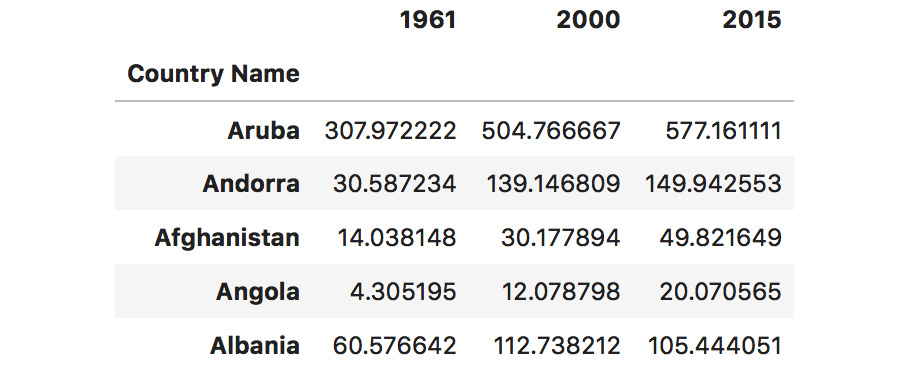Figure 1.48: Filtering data for 1961, 2000, and 2015

5. Use conditions to get all the countries that had a higher population density than `500` in `2000`. Simply pass this condition in brackets:
```"""
filtering countries that had a greater population density
than 500 in 2000
"""
dataset[(dataset["2000"] > 500)][["2000"]]```

The output of the preceding code is as follows: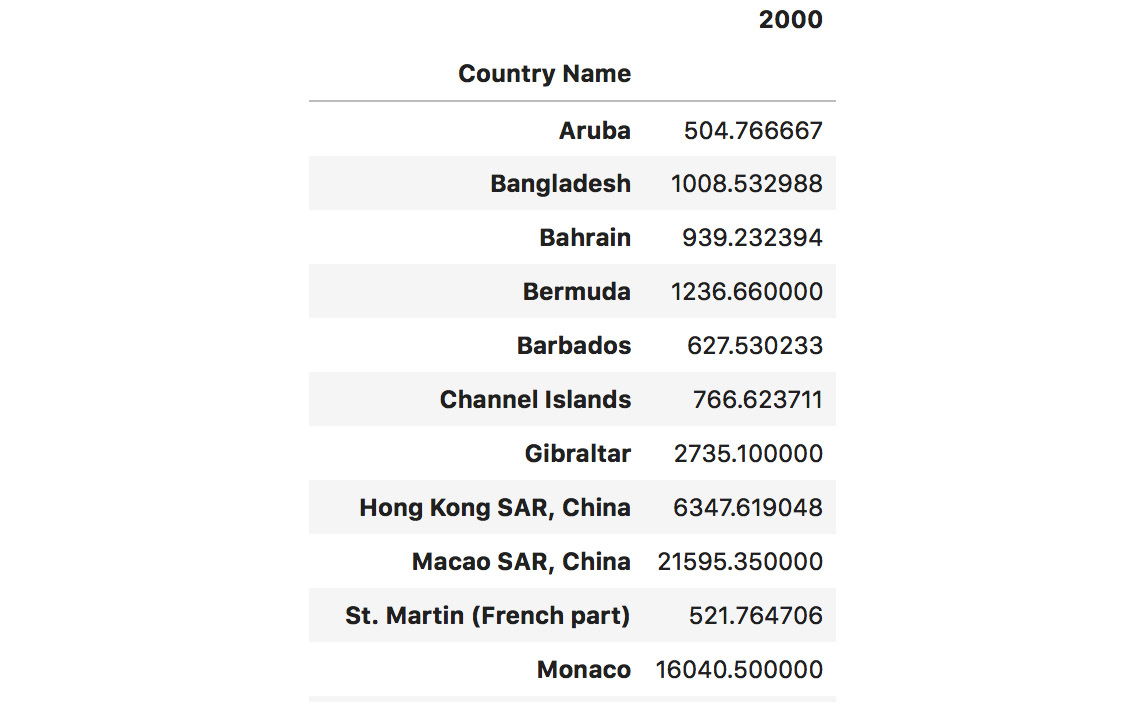Figure 1.49: Filtering out values that are greater than 500 in the 2000 column

6. Search for arbitrary columns or rows (depending on the index given) that match a certain `regex`. Get all the columns that start with 2 by passing `^2` (meaning that it starts at `2`):
`dataset.filter(regex="^2", axis=1).head()`

The output of the preceding code is as follows: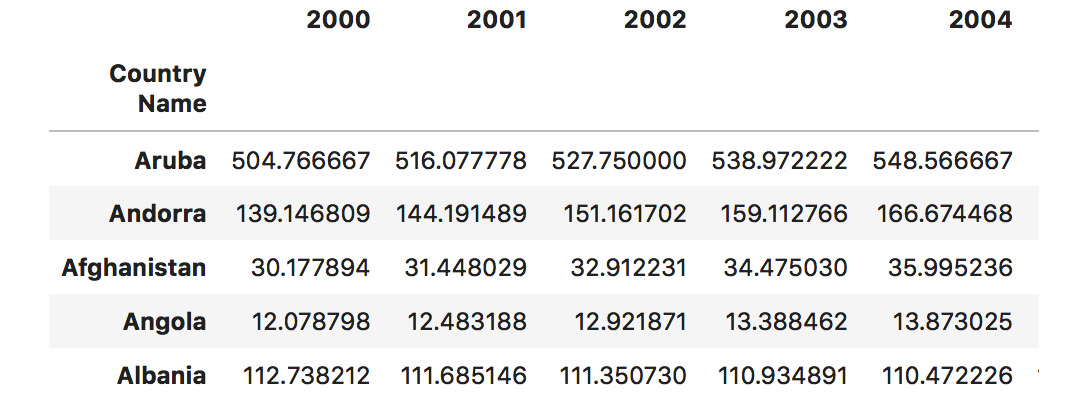Figure 1.50: Retrieving all columns starting with 2

7. Filter the rows instead of the columns by passing `axis=0`. This will be helpful for situations when we want to filter all the rows that start with `A`:
`dataset.filter(regex="^A", axis=0).head()`

The output of the preceding code is as follows: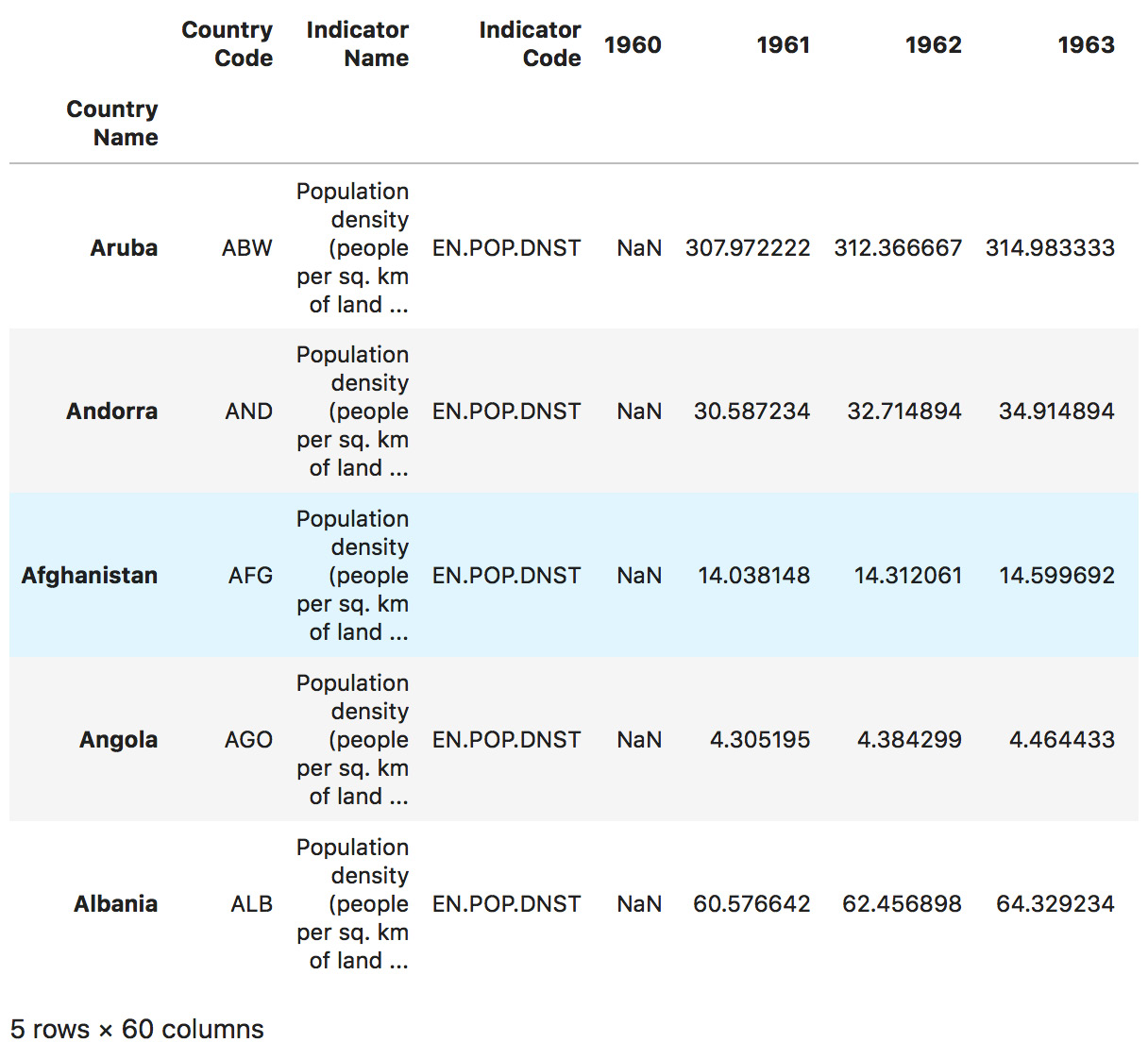8. Use the `like` query to find only the countries that contain the word `land`, such as Switzerland:
`dataset.filter(like="land", axis=0).head()`

The output of the preceding code is as follows: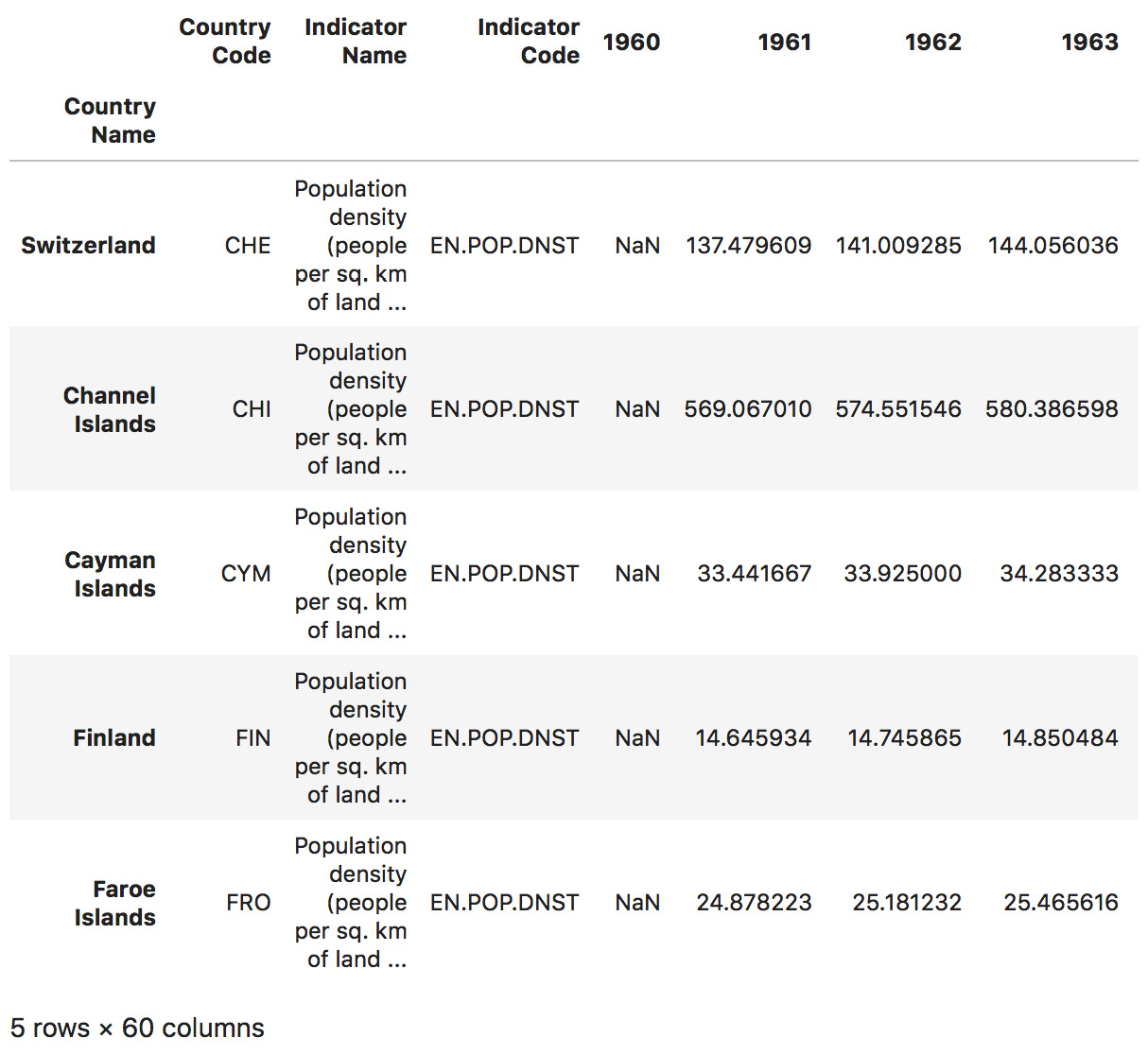Figure 1.52: Retrieving all countries containing the word "land"

Sorting

1. Use the `sort_values` or `sort_index` method to get the countries with the lowest population density for the year 1961:
`dataset.sort_values(by=["1961"])[["1961"]].head(10)`

The output of the preceding code is as follows: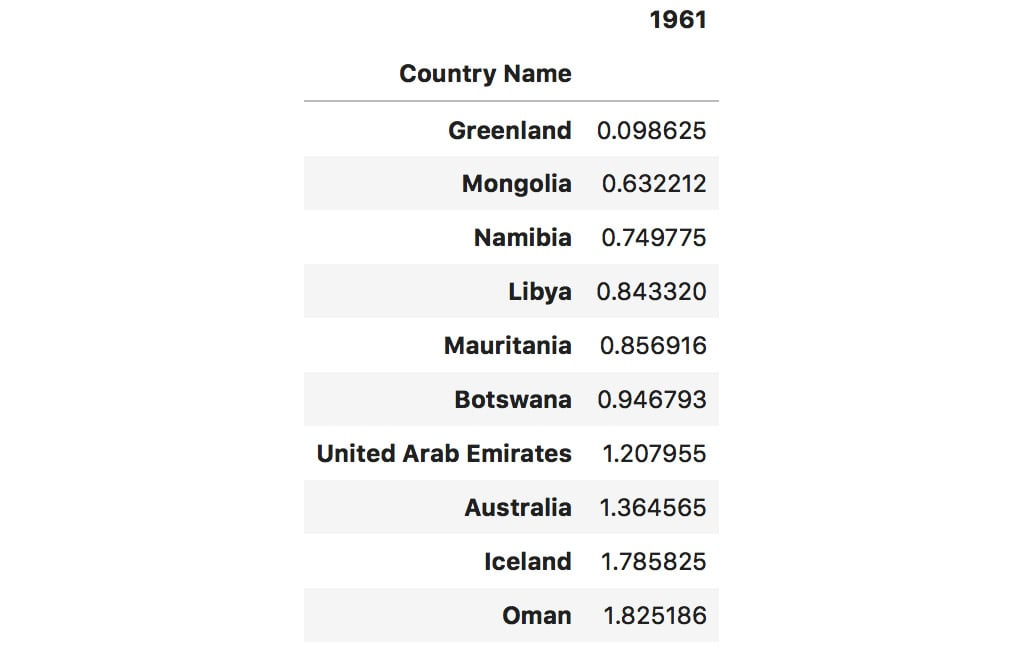Figure 1.53: Sorting by the values for the year 1961

2. Just for comparison, carry out sorting for `2015`:
`dataset.sort_values(by=["2015"])[["2015"]].head(10)`

The output of the preceding code is as follows: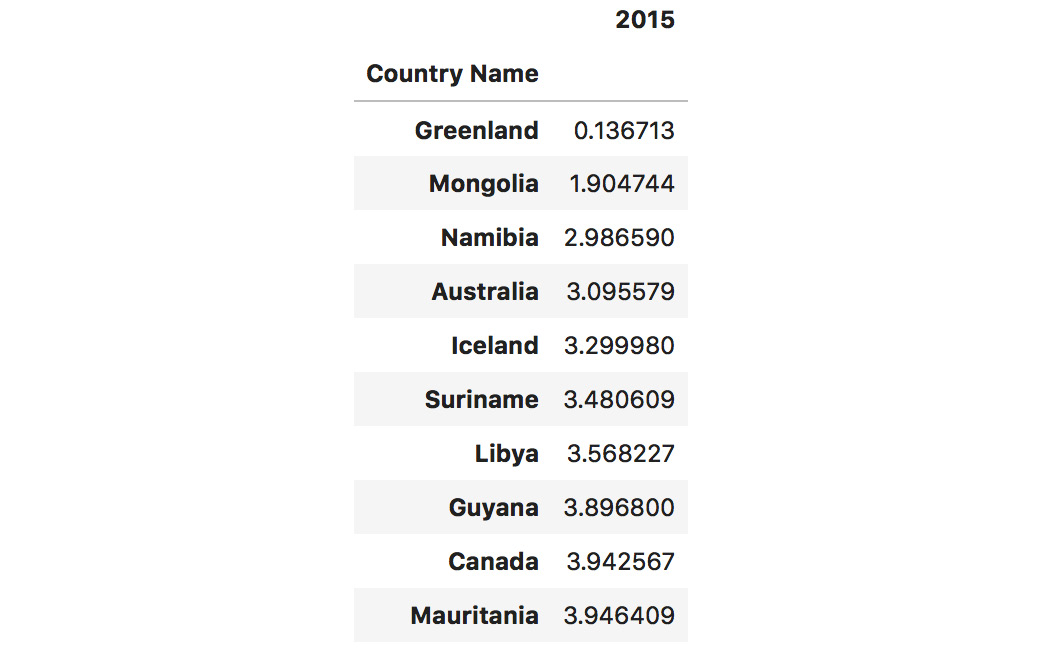Figure 1.54: Sorting based on the values of 2015

We can see that the order of the countries with the lowest population density has changed a bit, but that the first three entries remain unchanged.

3. Sort column 2015 in `descending` order to show the biggest values first:
```dataset.sort_values(by=["2015"], \

The output of the preceding code is as follows: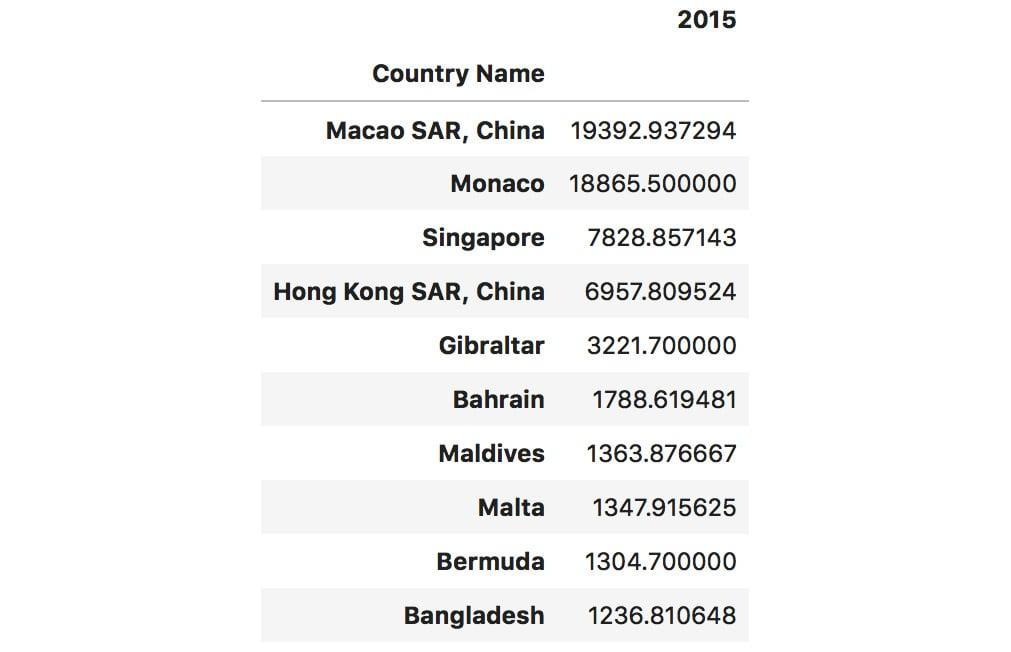Figure 1.55: Sorting in descending order

Reshaping

1. Get a DataFrame where the columns are `country codes` and the only row is the year `2015`. Since we only have one `2015` label, we need to duplicate it as many times as our dataset's length:
```# reshaping to 2015 as row and country codes as columns
dataset_2015 = dataset[["Country Code", "2015"]]
dataset_2015.pivot(index=["2015"] * len(dataset_2015), \
columns="Country Code", values="2015")```

The output of the preceding code is as follows: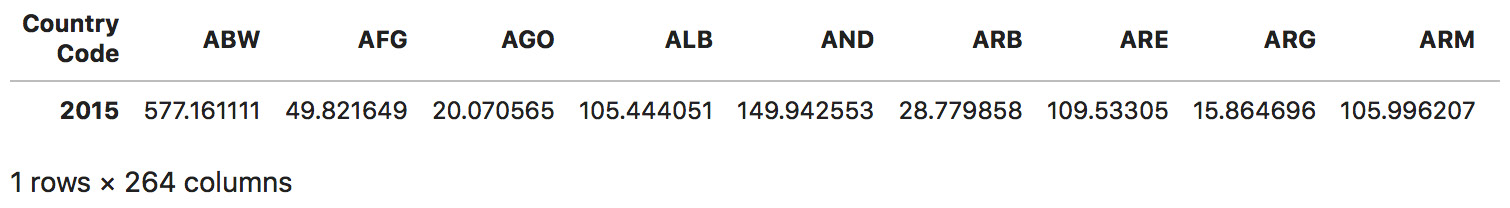Figure 1.56: Reshaping the dataset into a single row for the values of 2015

Note

To access the source code for this specific section, please refer to https://packt.live/2N0xHQZ.

You can also run this example online at https://packt.live/30Jeziw.

You now know the basic functionality of pandas and have already applied it to a real-world dataset. In the final activity for this chapter, we will try to analyze a forest fire dataset to get a feeling for mean forest fire sizes and whether the temperature of each month is proportional to the number of fires.

## Activity 1.02: Forest Fire Size and Temperature Analysis

In this activity, we will use pandas features to derive some insights from a forest fire dataset. We will get the mean size of forest fires, what the largest recorded fire in our dataset is, and whether the amount of forest fires grows proportionally to the temperature in each month.

Our forest fires dataset has the following structure:

• `X`: X-axis spatial coordinate within the Montesinho park map: 1 to 9
• `Y`: Y-axis spatial coordinate within the Montesinho park map: 2 to 9
• `month`: Month of the year: 'jan' to 'dec'
• `day`: Day of the week: 'mon' to 'sun'
• `FFMC`: FFMC index from the FWI system: 18.7 to 96.20
• `DMC`: DMC index from the FWI system: 1.1 to 291.3
• `DC`: DC index from the FWI system: 7.9 to 860.6
• `ISI`: ISI index from the FWI system: 0.0 to 56.10
• `temp`: Temperature in degrees Celsius: 2.2 to 33.30
• `RH`: Relative humidity in %: 15.0 to 100
• `wind`: Wind speed in km/h: 0.40 to 9.40
• `rain`: Outside rain in mm/m2: 0.0 to 6.4
• `area`: The burned area of the forest (in ha): 0.00 to 1090.84

Note

We will only be using the `month`, `temp`, and `area` columns in this activity.

The following are the steps for this activity:

1. Open the `Activity1.02.ipynb` Jupyter Notebook from the `Chapter01` folder to complete this activity. Import `pandas` using the `pd` alias.
2. Load the `forestfires.csv` dataset using pandas.
3. Print the first two rows of the dataset to get a feeling for its structure.

Derive insights from the sizes of forest fires

1. Filter the dataset so that it only contains entries that have an area larger than 0.
2. Get the mean, min, max, and std of the area column and see what information this gives you.
3. Sort the filtered dataset using the area column and print the last 20 entries using the `tail` method to see how many huge values it holds.
4. Then, get the median of the area column and visually compare it to the mean value.

Finding the month with the most forest fires

1. Get a list of unique values from the month column of the dataset.
2. Get the number of entries for the month of March using the `shape` member of our DataFrame.
3. Now, iterate over all the months, filter our dataset for the rows containing the given month, and calculate the mean temperature. Print a statement with the number of fires, the mean temperature, and the month.

Note

The solution for this activity can be found via this link.

You have now completed this topic all about pandas, which concludes this chapter. We have learned about the essential tools that help you wrangle and work with data. pandas is an incredibly powerful and widely used tool for wrangling and understanding data.

# Summary

NumPy and pandas are essential tools for data wrangling. Their user-friendly interfaces and performant implementation make data handling easy. Even though they only provide a little insight into our datasets, they are valuable for wrangling, augmenting, and cleaning our datasets. Mastering these skills will improve the quality of your visualizations.

In this chapter, we learned about the basics of NumPy, pandas, and statistics. Even though the statistical concepts we covered are basic, they are necessary to enrich our visualizations with information that, in most cases, is not directly provided in our datasets. This hands-on experience will help you implement the exercises and activities in the following chapters.

In the next chapter, we will focus on the different types of visualizations and how to decide which visualization would be best for our use case. This will give you theoretical knowledge so that you know when to use a specific chart type and why. It will also lay down the fundamentals of the remaining chapters in this book, which will focus on teaching you how to use Matplotlib and seaborn to create the plots we have discussed here. After we have covered basic visualization techniques with Matplotlib and seaborn, we will dive more in-depth and explore the possibilities of interactive and animated charts, which will introduce an element of storytelling into our visualizations.